• 第一个Matplotlib绘图程序
2022-02-17 12:21:12

# 第一个Matplotlib绘图程序

本节学习第一个 Matplotlib 绘图程序，如何使用 Matplotlib 绘制一个简单的折线图。下面绘制一个简单正弦曲线图，它显示了角度与正弦函数值之间的关系。

## 第一个绘图程序

首先导入 Matplotlib 包中的 Pyplot 模块，并以 as 别名的形式简化引入包的名称。

import matplotlib.pyplot as plt


接下来，使用 NumPy 提供的函数 arange() 创建一组数据来绘制图像。

#引入numpy包
import numpy as np
#获得0到2π之间的ndarray对象
x = np.arange(0, math.pi*2, 0.05)


上述所得 x 的值作用到 x 轴上，而该值对应的正弦值，也就是 y 值，使用以下方法获取：

y = np.sin(x)


使用 plot() 函数对 x、y 进行绘制。

plt.plot(x,y)


主要的绘图工作已经完成，不过还需要绘制一些细节，需要我们补充一下，比如图像的标题(title)、x 轴与 y 轴的标签（label）等。

plt.xlabel("angle")
plt.ylabel("sine")
plt.title('sine wave')
完整的程序代码如下：
from matplotlib import pyplot as plt
import numpy as np
import math
#调用math.pi方法弧度转为角度
x = np.arange(0, math.pi*2, 0.05)
y = np.sin(x)
plt.plot(x,y)
plt.xlabel("angle")
plt.ylabel("sine")
plt.title('sine wave')
#使用show展示图像
plt.show()


代码执行后，显示结果如下：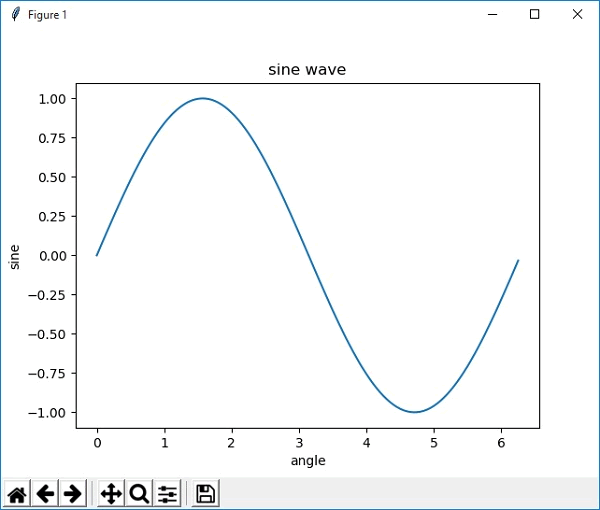图1：sine正弦函数图像

您也可以在 Jupyter 笔记本中运行 Matplotlib 的绘图程序。通过命令行或者开始菜单的方式启动 Jupyter 笔记本。启动成功后，将上述代码拷贝到输入行内，如下所示：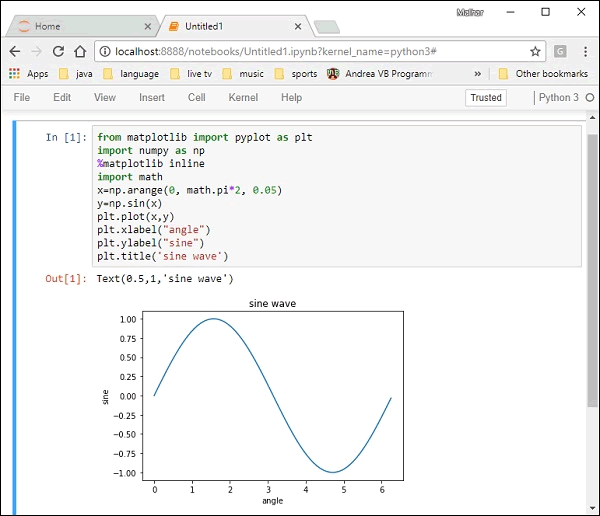注意：%matplotlib inline 是 Jupyter 提供的魔法命令，它可以把输出图显示在笔记本内部，否则会以查看器的形式单独显示。python 机器学习 深度学习 Matplotlib
更多相关内容
• 主要介绍了Python使用matplotlib绘图无法显示中文问题的解决方法,结合具体实例形式分析了Python使用matplotlib绘图时出现中文乱码的原因与相关解决方法,需要的朋友可以参考下
• 主要介绍了将matplotlib绘图嵌入pyqt的方法示例，文中通过示例代码介绍的非常详细，对大家的学习或者工作具有一定的参考学习价值，需要的朋友们下面随着小编来一起学习学习吧
• 利用python3请求zabbix_api接口，抓取某主机某监控项在某短时间的历史数据，并利用matplotlib绘制图形
• 作为数据分析的pandas库提供了Series DataFrame等类型的对象，我们也可以调用上述对象来绘图（如Series.plot()）。 本文将介绍这个库之间的关联，以及他们画图之间的异同。 1.关联 Pandas库提供了Series DataFrame...
• matplotlib 是python最著名的绘图库，它提供了一整套和matlab相似的命令API，十分...下面这篇文章主要给大家介绍了在python使用matplotlib绘图时图例显示问题的解决方法，需要的朋友可以参考学习，下面来一起看看吧。
• pycharm工程pyQt5使用matplotlib绘图，点击界面中的plot按钮实时更新绘图pyQt5
• 大家好，昨天我们讲解了如何使用Matplotlib官方文档来绘制并调整我们想要的图，那么今天将使用真实数据来练习使用Matplotlib绘图，我们开始吧！ 首先启动、导包、读取三连 import pandas as pd import numpy as ...

我的公众号：早起Python

===

大家好，昨天我们讲解了如何使用Matplotlib官方文档来绘制并调整我们想要的图，那么今天将使用真实数据来练习使用Matplotlib绘图，我们开始吧！

首先启动、导包、读取三连

import pandas as pd
import numpy as np
import matplotlib.pyplot as plt
import warnings
warnings.filterwarnings("ignore")

data = pd.read_csv('示例数据.csv')

看一下数据，就是一些国家不同年份的油价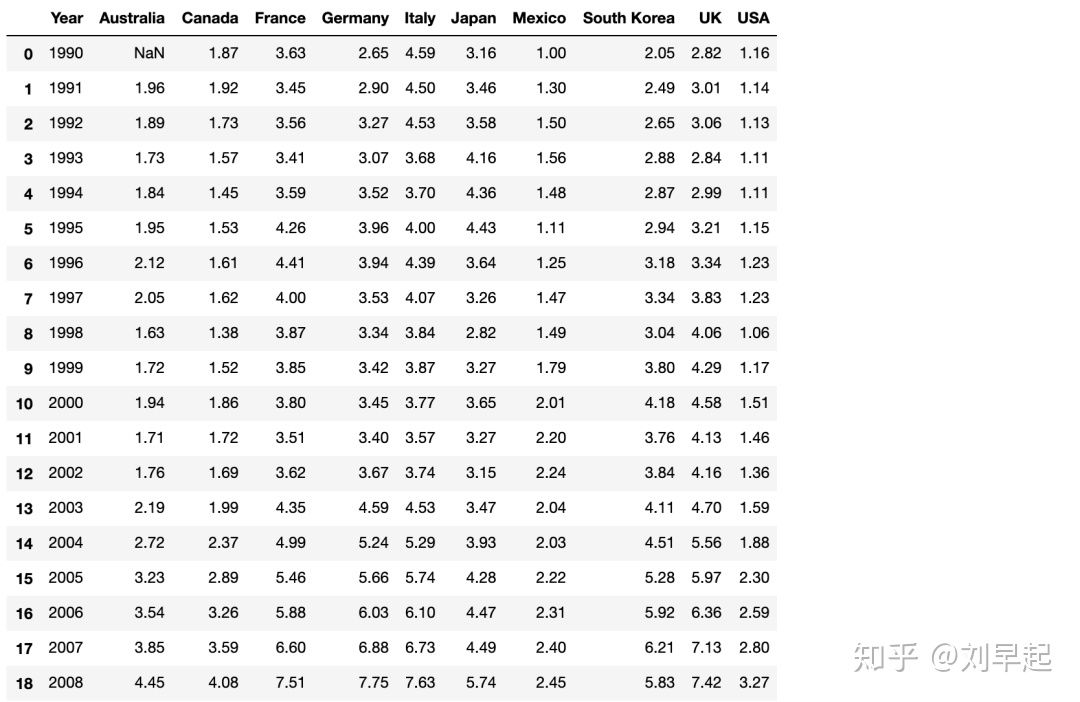接下来很自然的想法就是画个折线图看看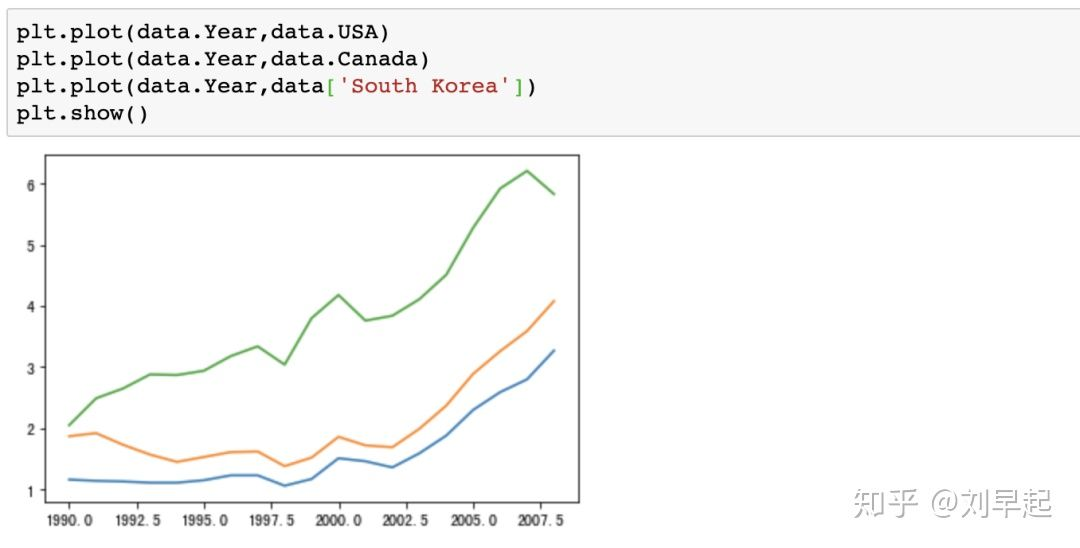好像少了点什么，对了，加个标题，并且就这图我也不知道哪根线对应哪个国家，所以需要再来个图例，方法和昨天文章一样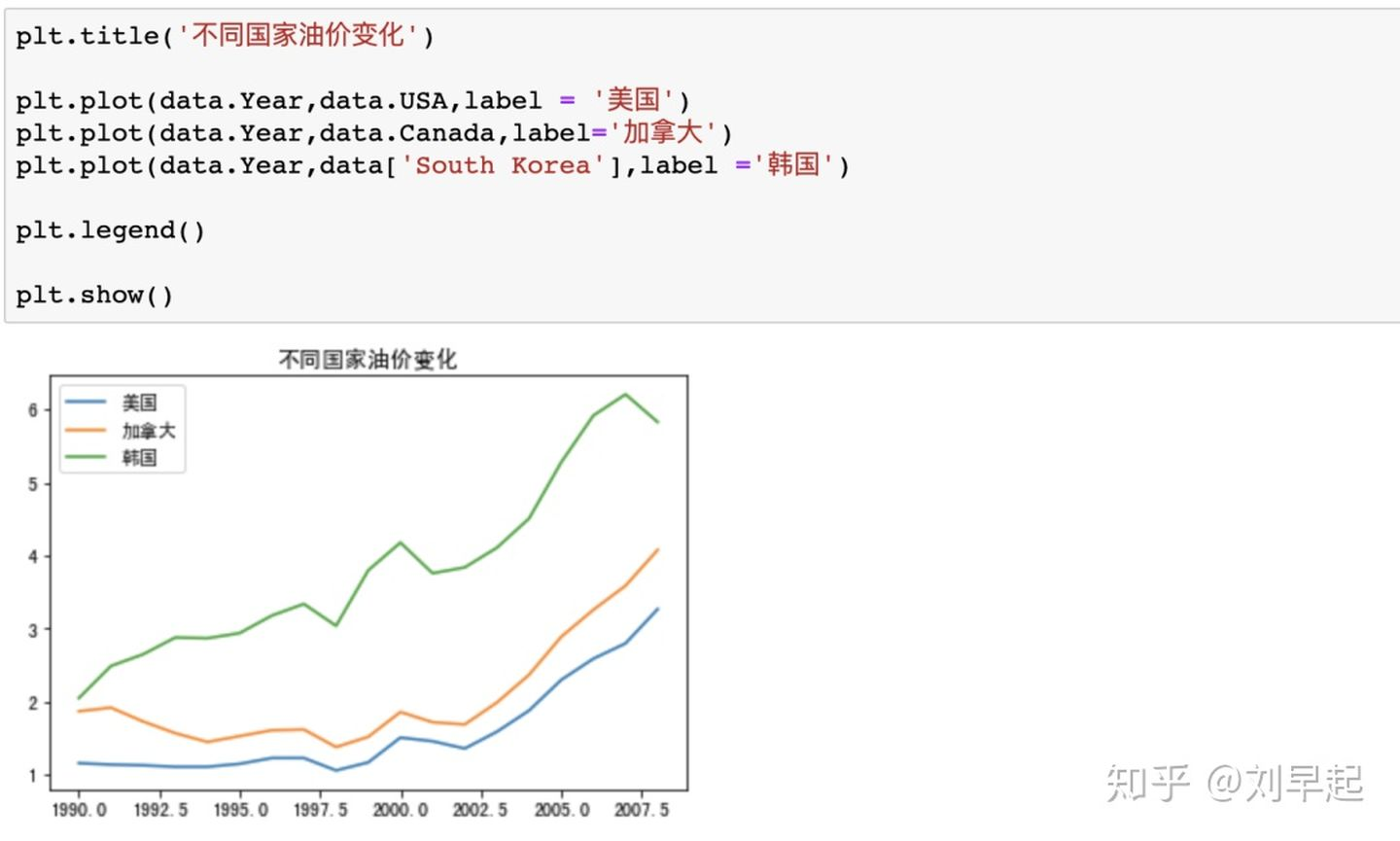好像图有点小，加一行代码让它变大点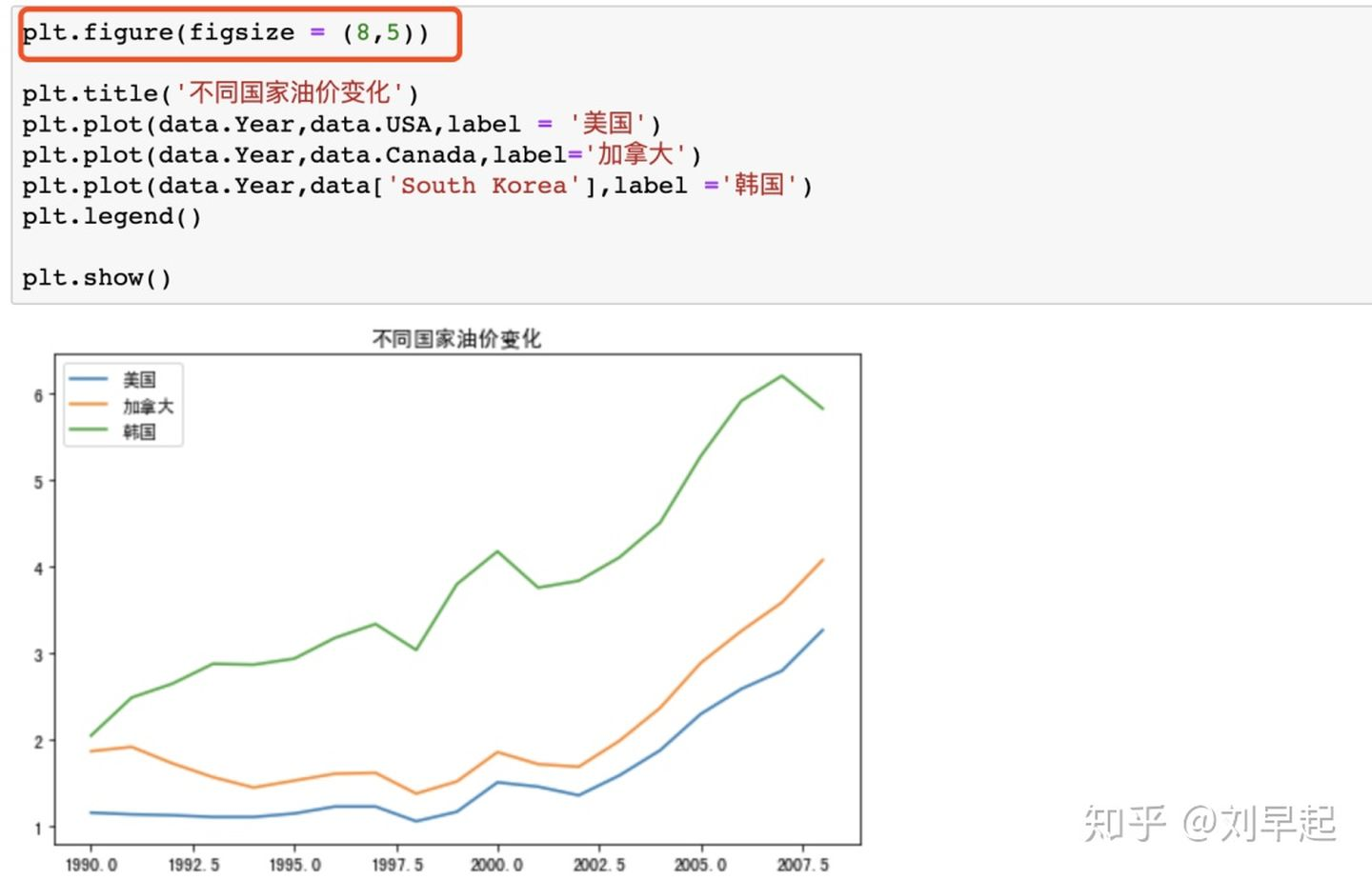接下来干嘛，咱们仔细看X轴，这年份还带小数点看的不难受吗，改之！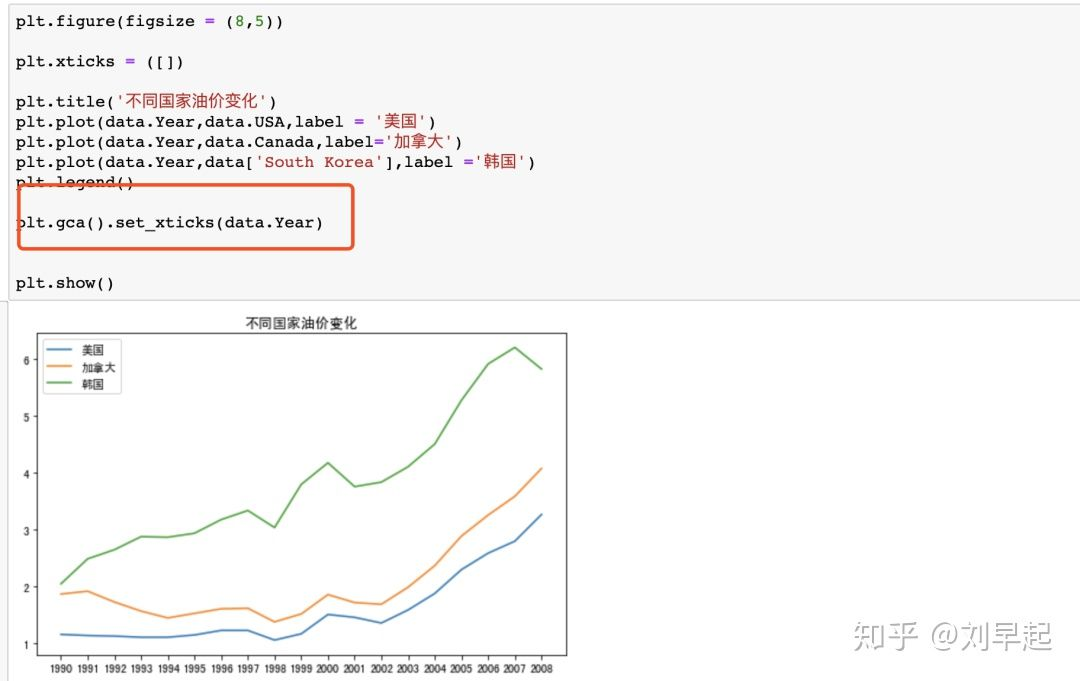现在x轴终于是正常的年份了，但是新的问题又来了，这X轴这么挤，我看的依旧难受，改之，每三年显示一个年份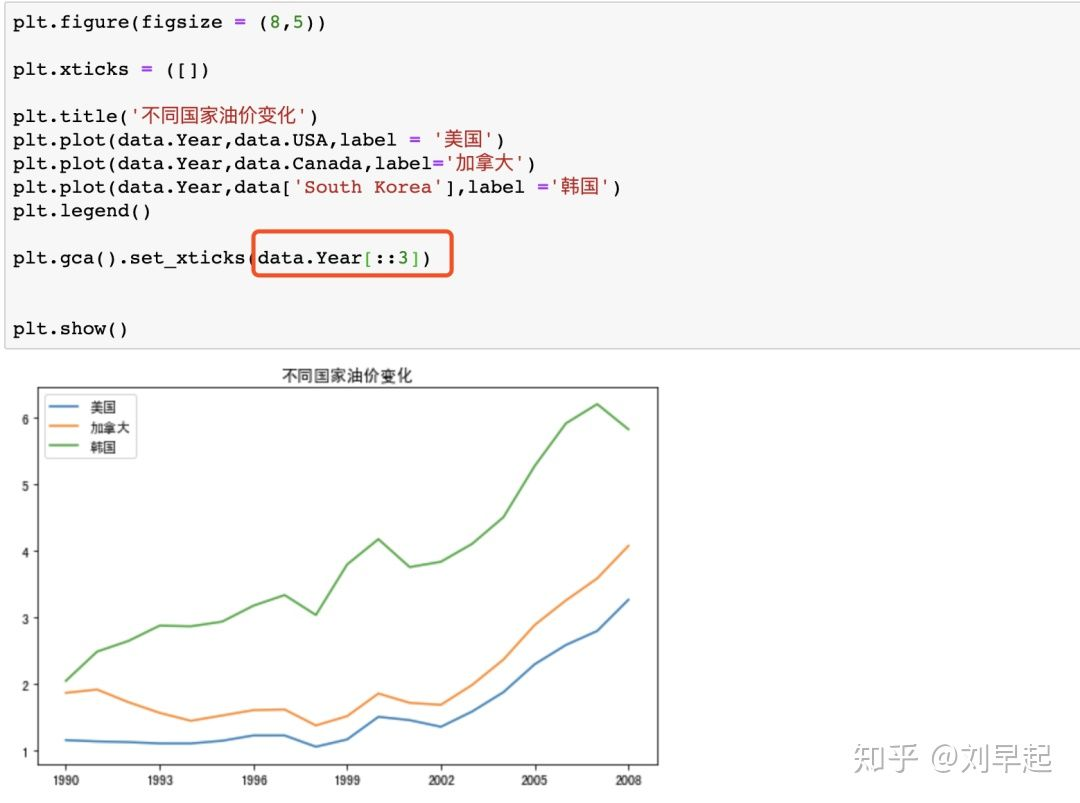接下来干嘛，总感觉这个图看的不爽，并且我想显示每一个点，所以再改改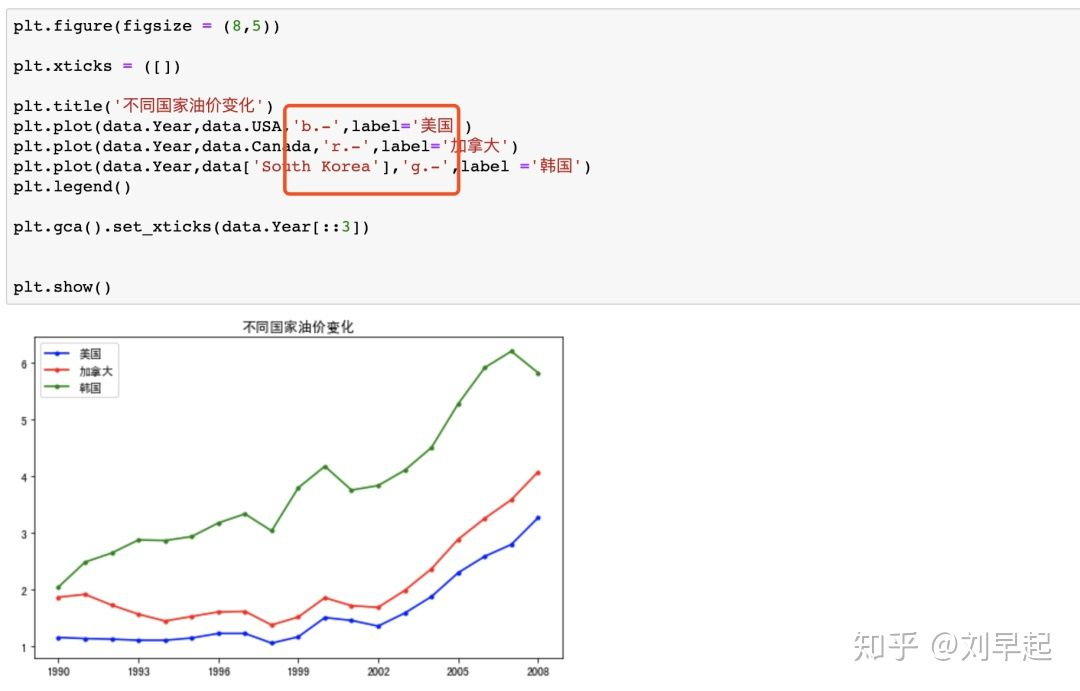感觉好多了，咦，我x轴y轴呢，安排！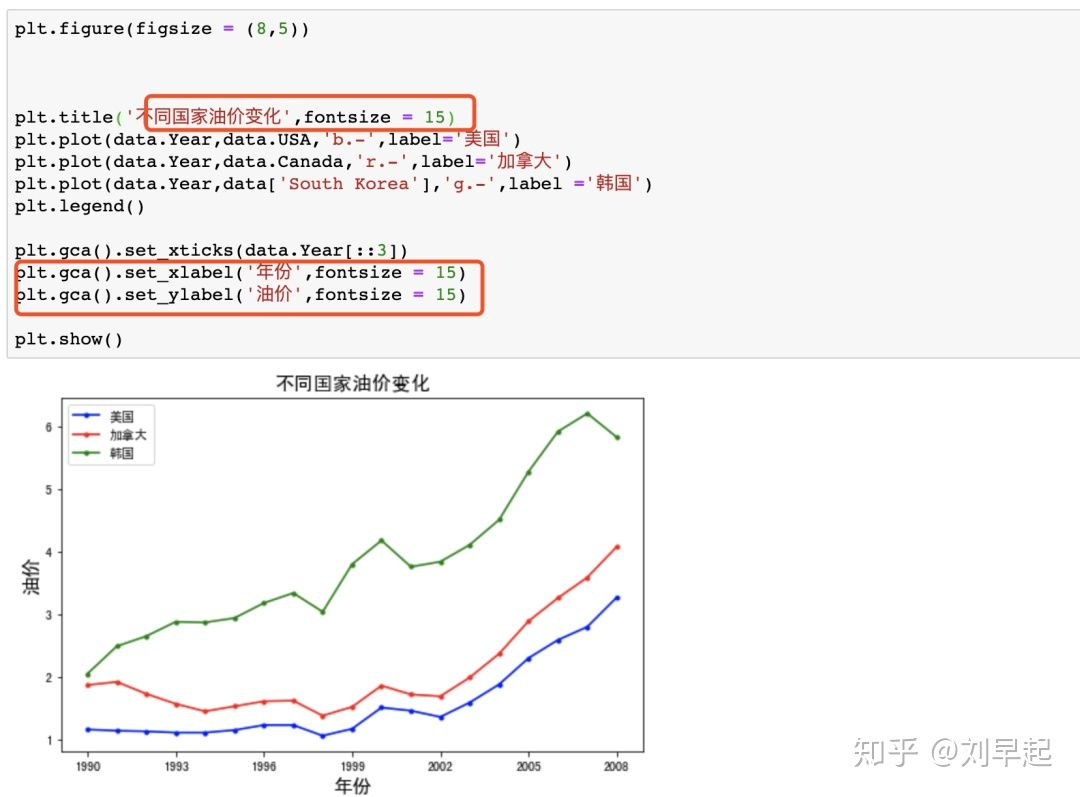好了，差不多了，早起基本满意，现在让我们来试着展示所有国家的数据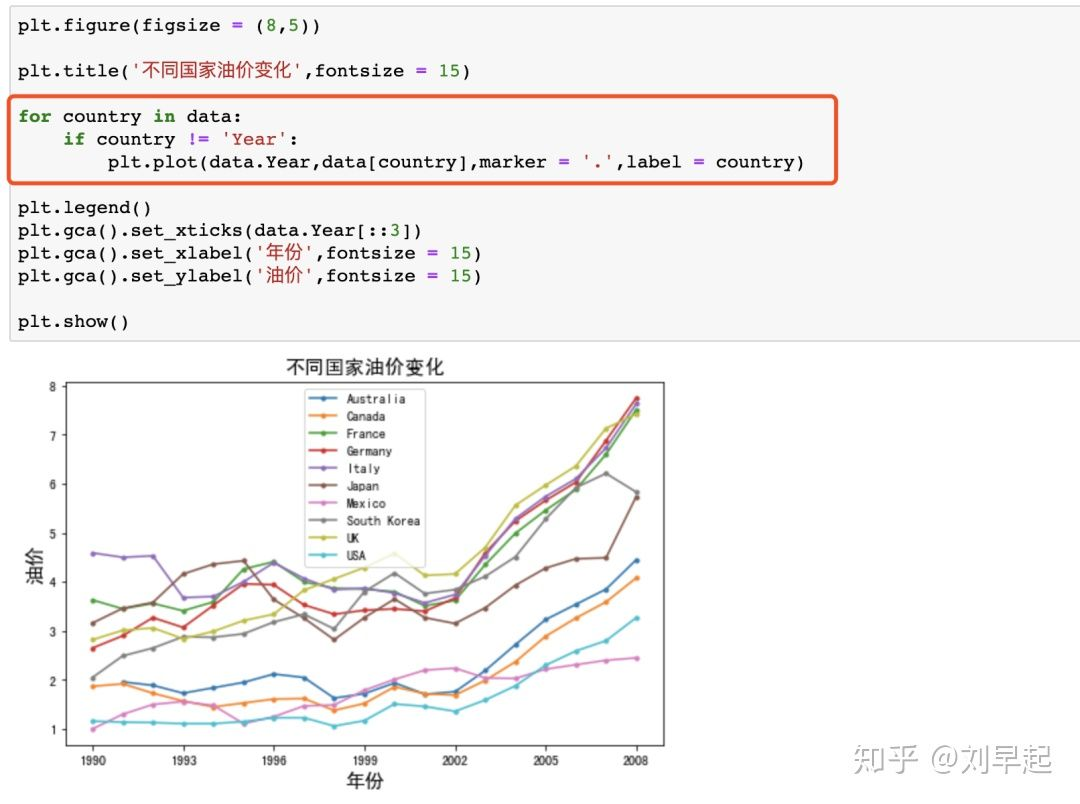不过这自动生成的图例是什么鬼，改之，并且国家这么多，图例放图中怎么都会挡住图，所以我还要给它整外面去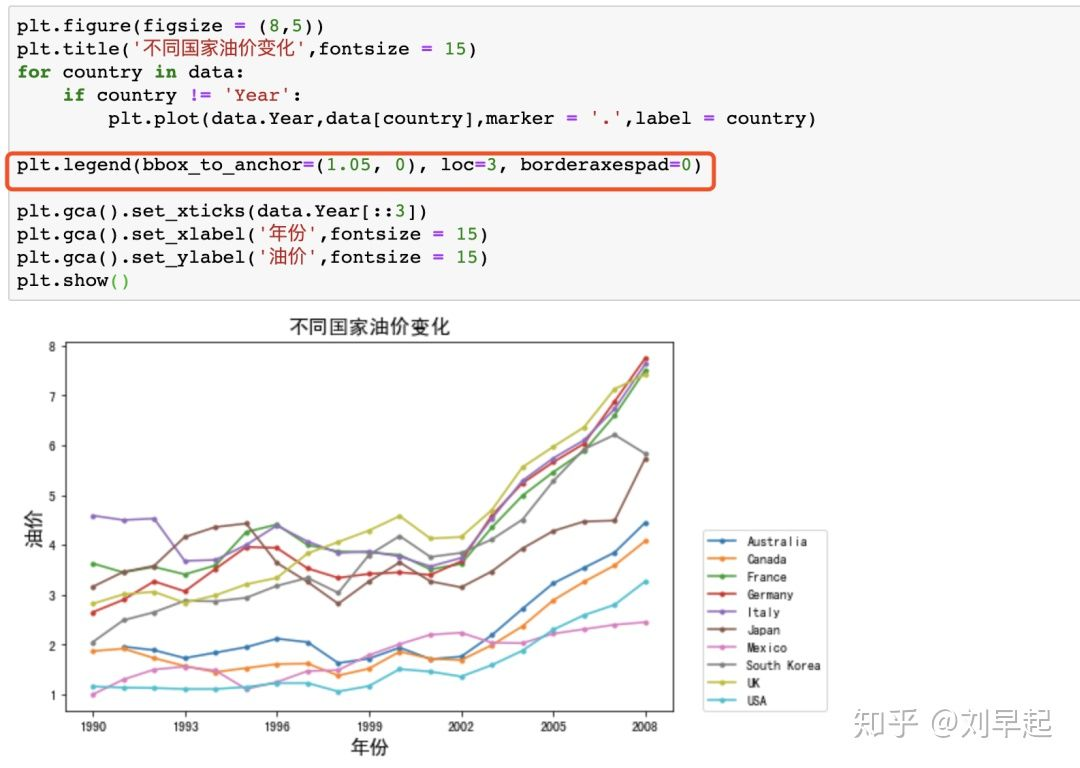OK，搞定，最后保存一下就算完成了我们第一份Matplotlib作业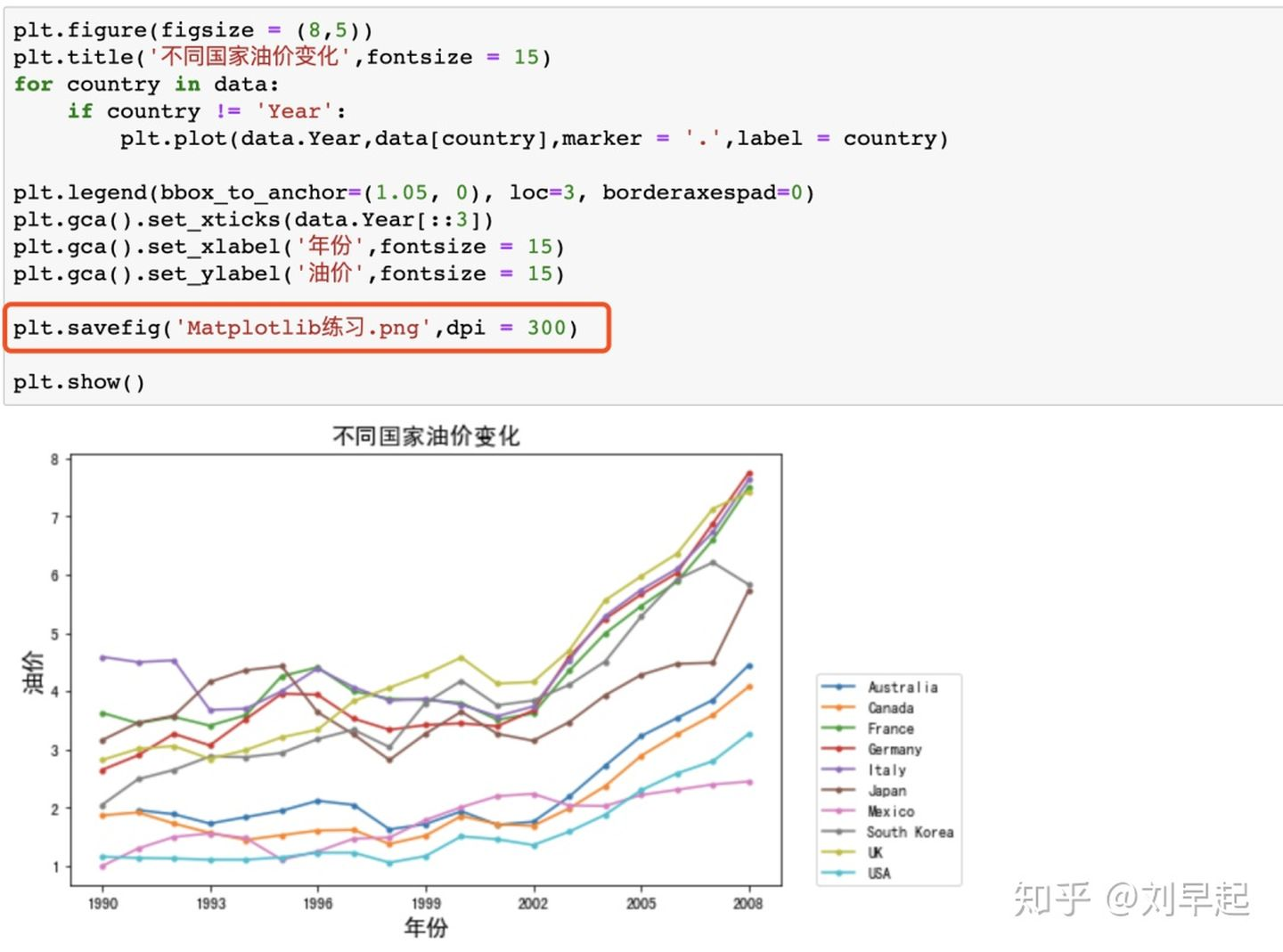展开全文• ## 使用Matplotlib绘图

千次阅读 2022-03-21 19:06:09
使用Matplotlib绘图，绘制多条线，线条的颜色、风格、标记等方法，绘制柱状图，多个柱状图显示，柱状图标注、颜色、图标等操作； plt.plot() plt.bar() plt.xlabel() plt.ylabel() plt.title() plt.grid() plt....

🤵 AuthorHorizon Max

编程技巧篇各种操作小结

🎇 机器视觉篇会变魔术 OpenCV

💥 深度学习篇简单入门 PyTorch

🏆 神经网络篇经典网络模型

💻 算法篇再忙也别忘了 LeetCode

## 使用Matplotlib绘图

1）： plt.plot() ：曲线函数

颜色
r : 红色 m : 洋红色
g : 绿色 y : 黄色
b : 蓝色 k : 黑色
w : 白色 c : 青绿色
#008000 : RGB某颜色

风格
‐ : 实线
‐‐ : 破折线
‐. : 点划线
: : 虚线
  : 无线条

标记
. : 点标记
, : 像素标记(极小点)
o : 实心圈标记
v : 倒三角标记
^ : 上三角标记
> : 右三角标记
< : 左三角标记

2）： plt.bar() ：柱状图

3）： plt.xlabel() ：坐标

4）： plt.title() ：图表标题

5）： plt.grid() ：网格显示

6）： plt.legend() ：图例

0 ---- best自己分配最佳位置
1 ---- upper right
2 ---- upper left
3 ---- lower left
4 ---- lower right
5 ---- right
6 ---- center left
7 ---- center right
8 ---- lower center
9 ---- upper center
10 ---- center

plt.legend(loc=7, frameon=False, ncol=1, fontsize=8, framealpha=0.5)
loc=7 ：图列位置 = ’ center right ’
frameon=False ：边框是否显示
ncol=2 ：图例分栏
fontsize=8 ：字体大小
framealpha=0.5 ：图例透明度

## 示例1——曲线图

import numpy as np
import matplotlib.pyplot as plt

x = np.linspace(0,2,100)
y1 = x
y2 = x**2
y3 = x**3

plt.plot(x, y1, 'r-', label='linear')
plt.plot(x, y3, 'b--', label='cubic')

plt.xlabel('x')
plt.ylabel('y')
plt.title('Example for draw lines')

plt.grid(True)
plt.legend(loc='upper right')
plt.show()


输出结果：## 示例2——柱状图

import matplotlib.pyplot as plt
import matplotlib as mpl
import numpy as np

def draw(acc1, acc2, acc3, acc4):
mpl.rcParams["font.sans-serif"] = ["SimHei"]
mpl.rcParams["axes.unicode_minus"] = False

x = np.arange(4)

bar_width = 0.15
tick_label = ['Model1', 'Model2', 'Model3', 'Model4']
plt.figure(figsize=(10, 6))
plt.bar(x-3*bar_width/2, acc1, bar_width, align="center", color='orange', label='acc1', alpha=0.5)
plt.bar(x-bar_width/2, acc2, bar_width, color='m', align="center", label='acc2', alpha=0.5)
plt.bar(x+bar_width/2, acc3, bar_width, color='b', align="center", label='acc3', alpha=0.5)
plt.bar(x+3*bar_width/2, acc4, bar_width, color='r', align="center", label='acc4', alpha=0.5)
for j in range(4):
fontsize=8, color='#EE00EE', ha='center', va='bottom')
plt.text(j-bar_width/2, acc2[j], str('{:.2f}'.format(test_acc[j]) + '%'),
fontsize=8, color='#EE00EE', ha='center', va='bottom')
plt.text(j+bar_width/2, acc3[j], str('{:.2f}'.format(train_acc[j]) + '%'),
fontsize=8, color='#EE00EE', ha='center', va='bottom')
plt.text(j+3*bar_width/2, acc4[j], str('{:.2f}'.format(clean_acc[j]) + '%'),
fontsize=8, color='#EE00EE', ha='center', va='bottom')

plt.title('Models and Accuracy')
plt.xticks(x, tick_label)
plt.xlabel("Models")
plt.ylabel("Accuracy")
plt.legend(loc=2, frameon=False, fontsize=8, framealpha=0.5)
plt.show()

acc1 = [18.630, 90.050, 5.110, 89.850]
acc2 = [85.310, 91.790, 85.310, 90.480]
acc3 = [53.258, 97.873, 45.701, 96.580]
acc4 = [97.730, 91.066, 97.730, 88.822]
draw(acc1, acc2, acc3, acc4)


输出结果：展开全文python plt
• 一、Matplotlib绘图的编程方式： 1、pyplot： 是 Matplotlib 的子库，提供了和 MATLAB 类似的绘图 API。（常用） 2、pylab：将Matplotlib...1、Matplotlib绘图标记使用plot()方法的marker参数定义 2、Matplotlib绘图线

# 目录

一、Matplotlib绘图的编程方式：

1、pyplot： 是 Matplotlib 的子库，提供了和 MATLAB 类似的绘图 API。（常用）

2、pylab：将Matplotlib和Numpy合并的模块，模拟Matlab的编程环境。（不推荐使用）

3、面向对象的方式：Matplotlib的精髓，更基础和底层的方式。（常用）

二、Matplotlib绘图基础

1、Matplotlib绘图标记使用plot()方法的marker参数定义

2、Matplotlib绘图线

（1）Matplotlib线的类型用linestyle参数来定义：

（2）Matplotlib线的颜色用color参数来定义

（3）Matplotlib线的宽度用linewidth参数来定义

（4）Matplotlib轴标签使用xlabel()和ylabel()方法

（5）Matplotlib标题使用title()方法

（6）Matplotlib标题与标签的定位提供了loc参数

（7）Matplotlib网格线使用pyplot中的grid()方法

（8）绘制多图使用pyplot中的subplot()和subplots()方法

# 一、Matplotlib绘图的编程方式：

## 1、pyplot： 是 Matplotlib 的子库，提供了和 MATLAB 类似的绘图 API。（常用）

import matplotlib.pyplot as plt
import numpy as np
x=np.arange(0,10)
y=np.random.randn(len(x))
plt.plot(x,y)
plt.title('plplot')
plt.show()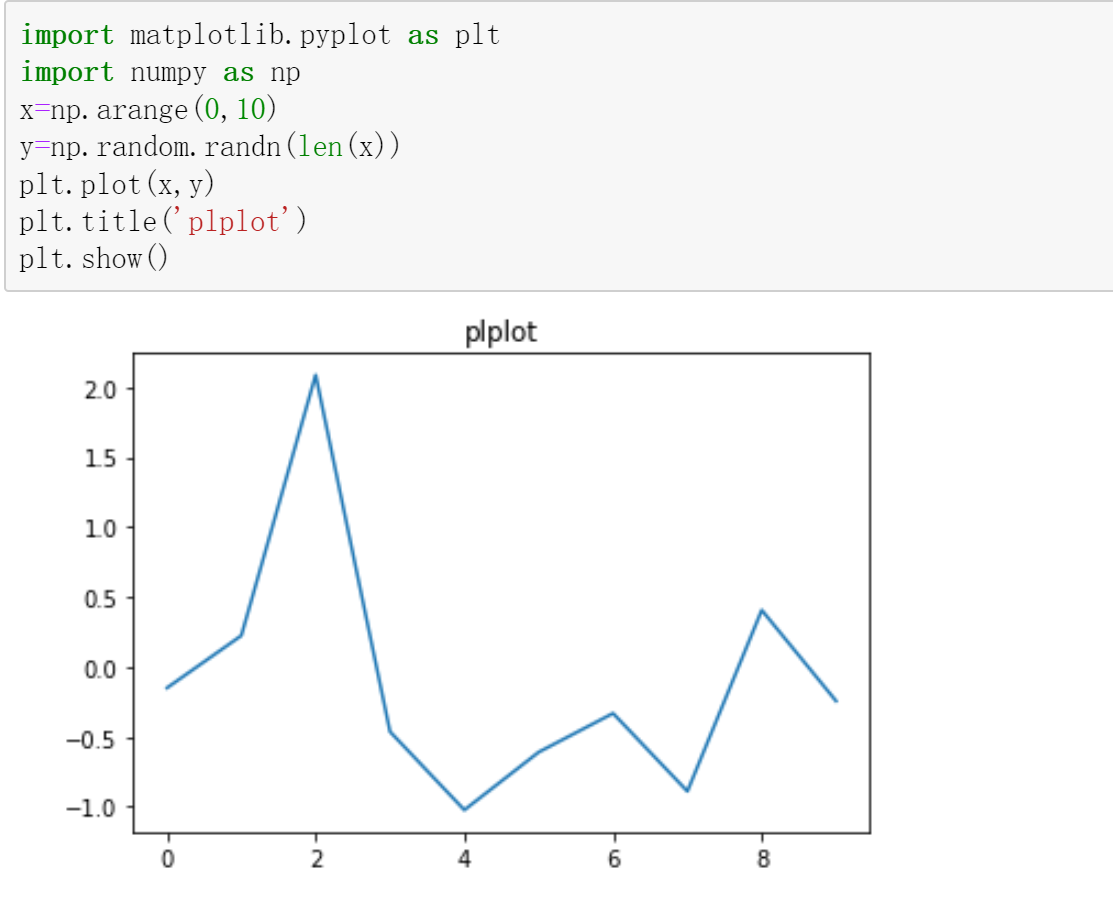## 2、pylab：将Matplotlib和Numpy合并的模块，模拟Matlab的编程环境。（不推荐使用）

from pylab import *
x=arange(0,10)
y=randn(len(x))
plot(x,y)
title('pylab',size=15)
show()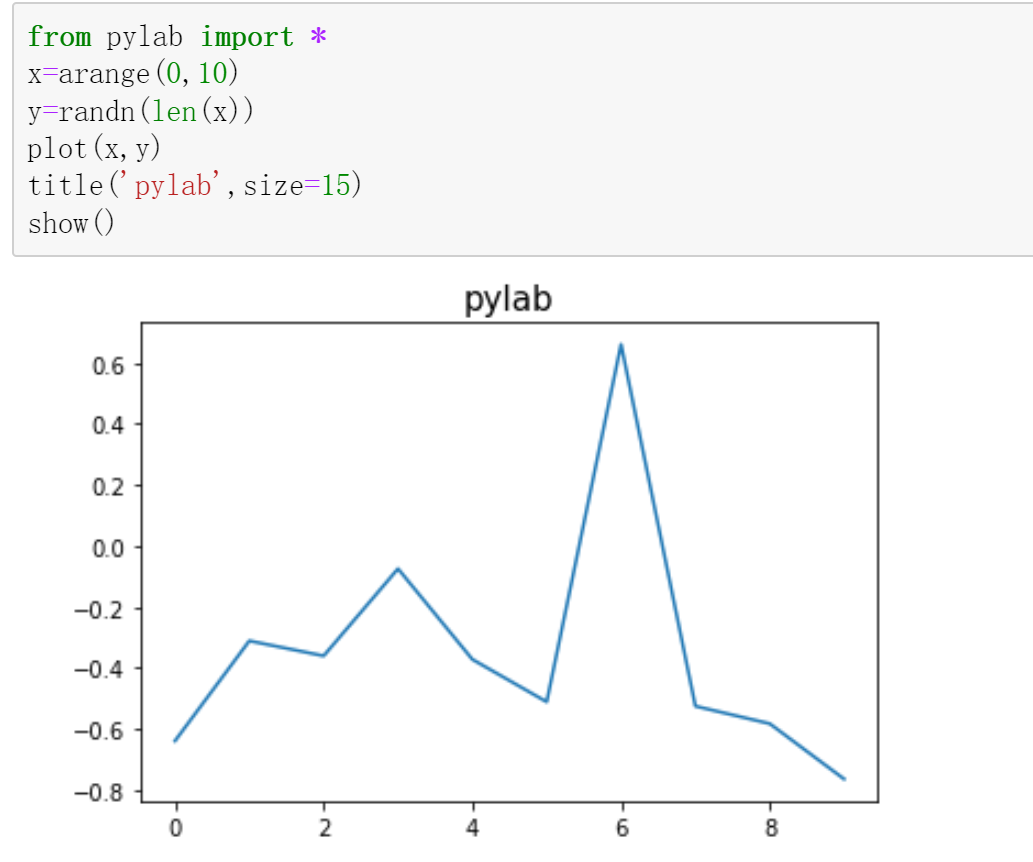总结pyplot与pylab的区别：从pylab代码可以看出，通过pylab可以直接调用函数。例：arange(0,10)，而不是np.arange(0,10)

## 3、面向对象的方式：Matplotlib的精髓，更基础和底层的方式。（常用）

import matplotlib.pyplot as plt
import numpy as np

x=np.arange(0,10)

y=np.random.randn(len(x))

fig=plt.figure()

l,=plt.plot(x,y)

t=ax.set_title('object')

plt.show()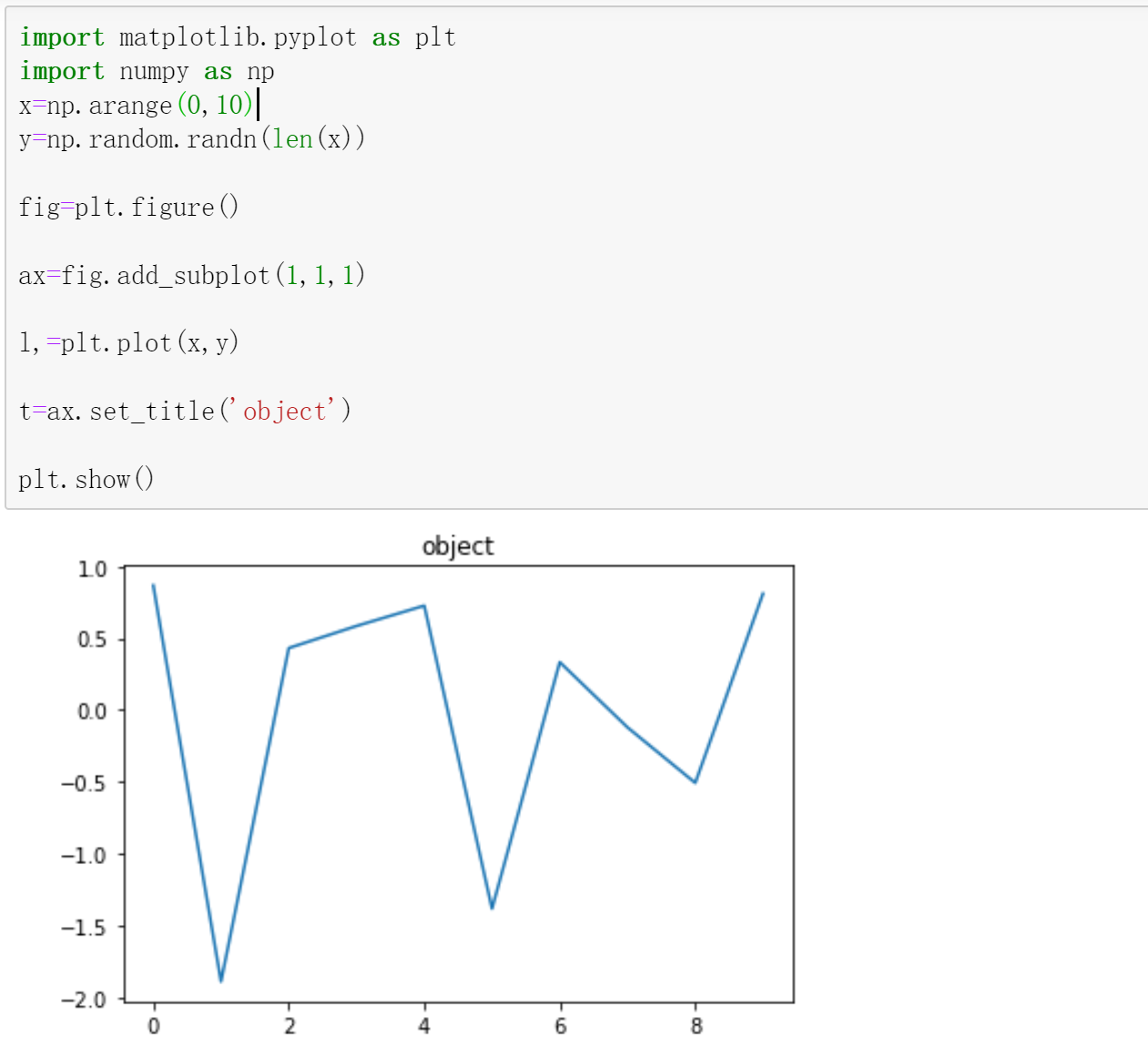总结：使用面向对象编程，需要画布(FigureCanvas)、图像(Figure)、坐标轴(Axes)

 编程方式 优势 劣势 pyplot 简单易用，交互使用方便，可以根据命令实时作图 底层定制能力不足 pylab 完全封装，环境最接近Matlab 和matplotlib设计理念不太一致，不利于学习matplotlib 面向对象 接近Matplotlib基础和底层的方式 9难度稍大

实战中，根据需求，综合使用pyplot和面向对象的方式。

# 二、Matplotlib绘图基础

## 1、Matplotlib绘图标记使用plot()方法的marker参数定义

merker样式参考：

Matplotlib marker参数样式大全_小羊快学的博客-CSDN博客一、样式大全标记类型标记样式效果o实心圆.点,像素点+加号*星号x乘号X填充乘号s正方形D胖菱形d瘦菱形p五边形h六边形H六边形8八边形^上三角v...
https://blog.csdn.net/Yangyuqing_/article/details/124099762?spm=1001.2014.3001.5502

import matplotlib.pyplot as plt
import numpy as np

x=np.arange(0,10)

plt.plot(x,marker='+')

plt.show()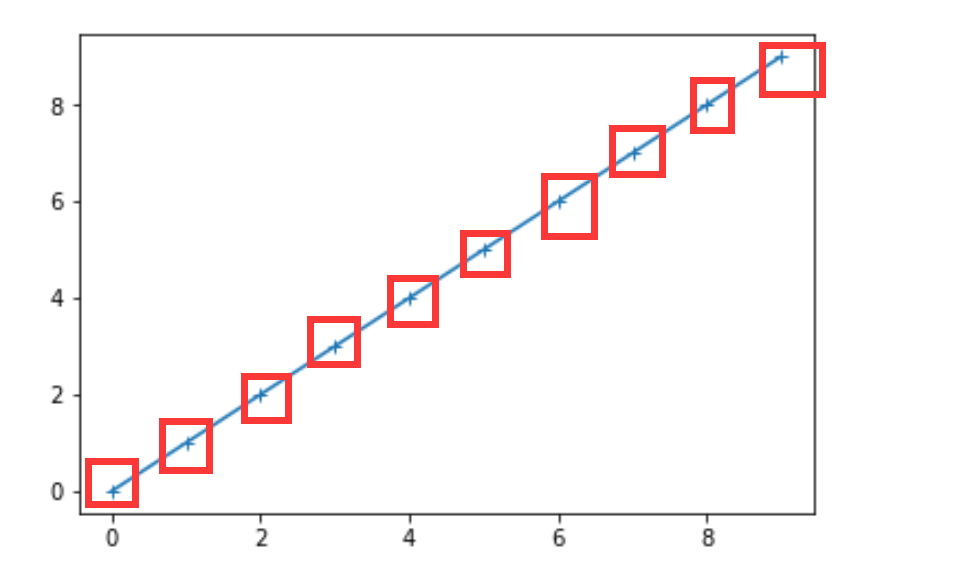## 2、Matplotlib绘图线

定义线的类型、颜色与大小等

### （1）Matplotlib线的类型用linestyle参数来定义：

 类型(简写) 说明 '-' 实线 ':' 点虚线 '--' 破折线 '-.' 点划线 ''或' ' 不画线 'steps' 阶梯线
import matplotlib.pyplot as plt
import numpy as np

x=np.arange(0,10)

plt.plot(x,marker='*',linestyle=':')

plt.show()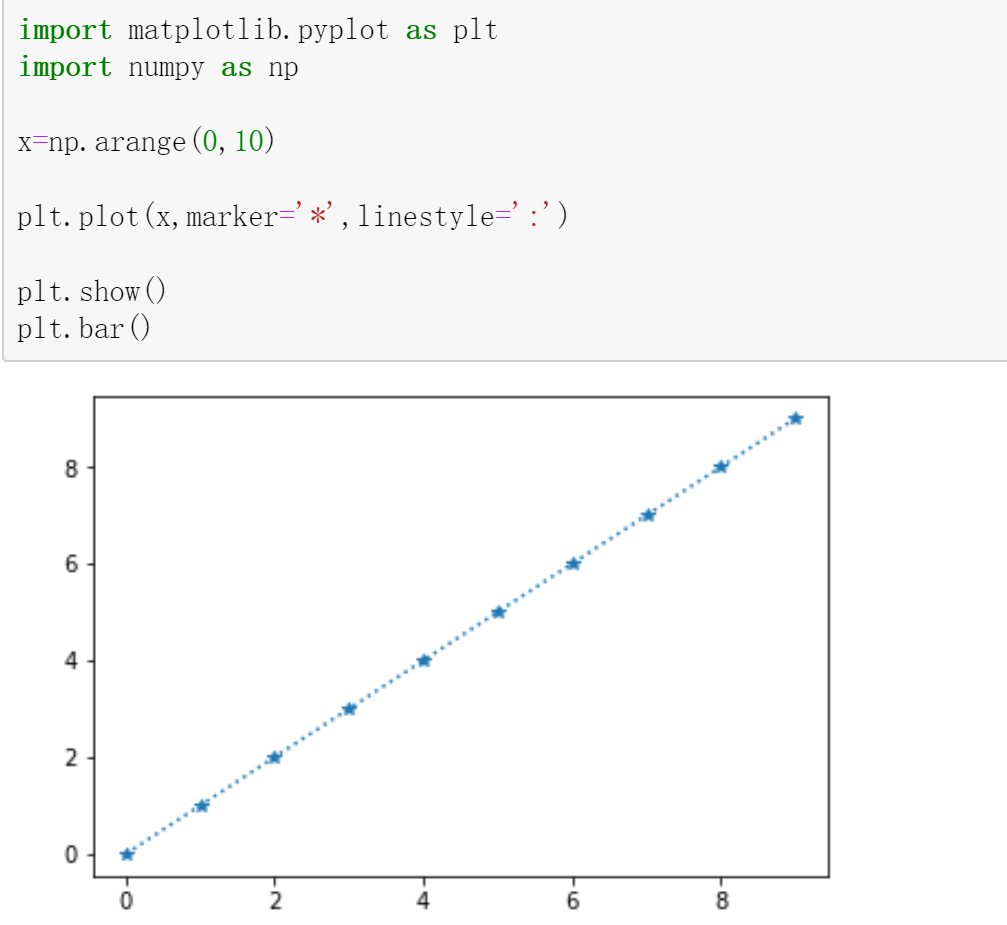### （2）Matplotlib线的颜色用color参数来定义

 颜色标记 描述 'r' 红色 'g' 绿色 'c' 青色 'y' 黄色 'k' 黑色

说明：列举不全，完整样式参考颜色值

import matplotlib.pyplot as plt
import numpy as np

x=np.arange(0,10)

plt.plot(x,marker='*',linestyle=':',color='r')

plt.show()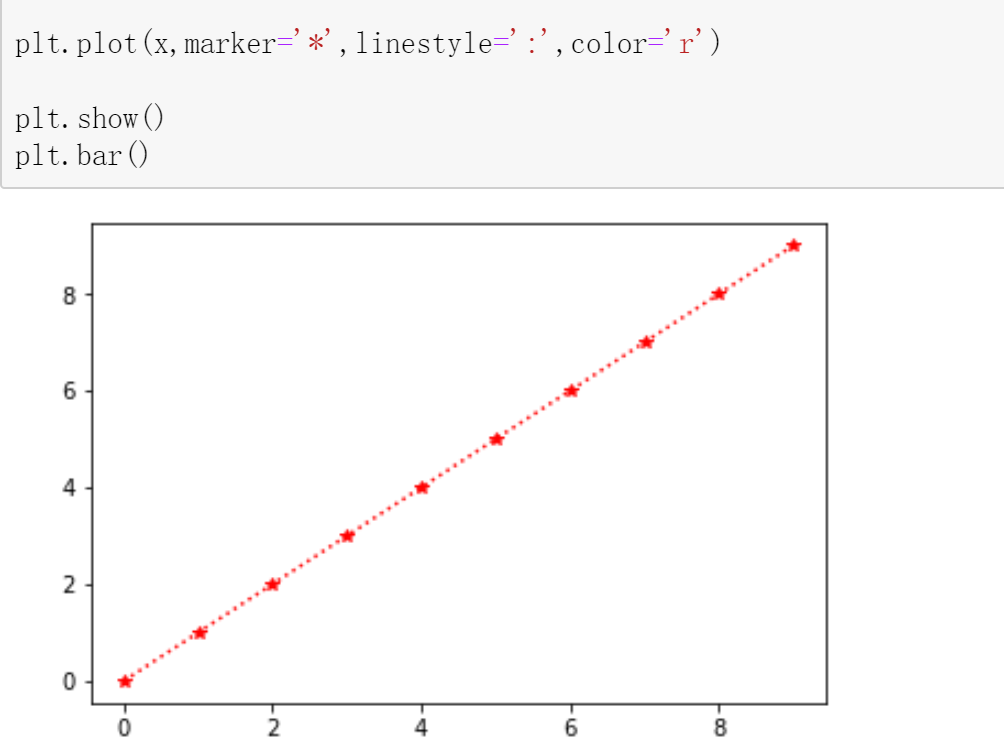### （3）Matplotlib线的宽度用linewidth参数来定义

说明：值除了是整数，也可以是浮点数。

import matplotlib.pyplot as plt
import numpy as np

x=np.arange(0,10)

plt.plot(x,marker='*',linestyle=':',color='r',linewidth=4.5)

plt.show()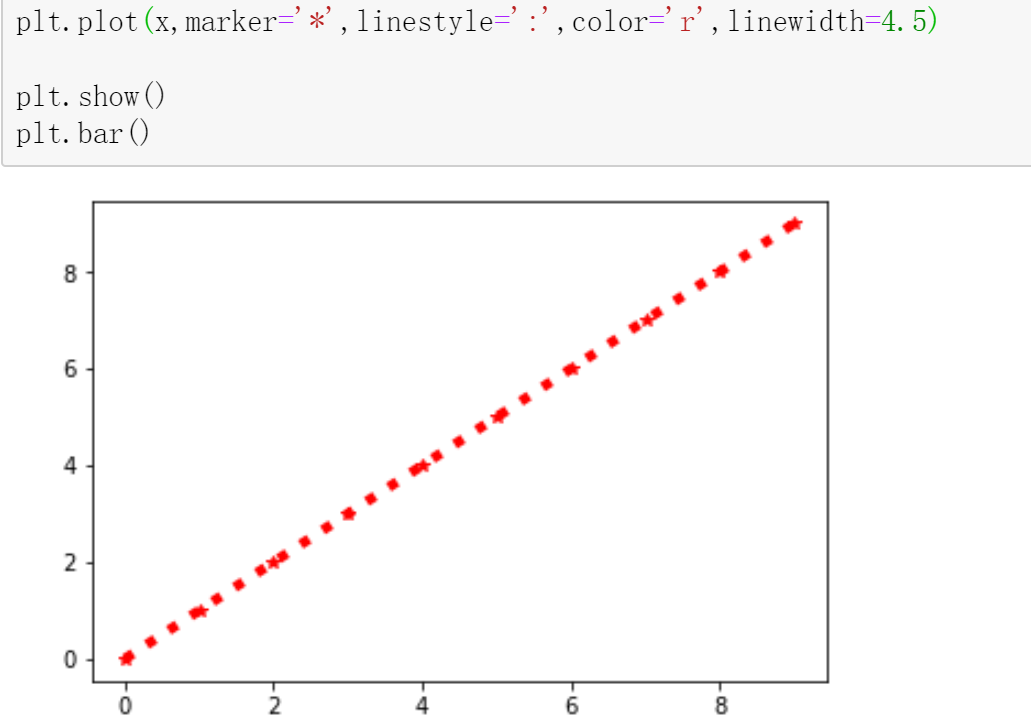### （4）Matplotlib轴标签使用xlabel()和ylabel()方法

import matplotlib.pyplot as plt
import numpy as np

x=np.arange(0,10)

plt.plot(x,marker='*',linestyle=':',color='r',linewidth=4.5)
plt.xlabel('x-label')
plt.ylabel('y-label')

plt.show()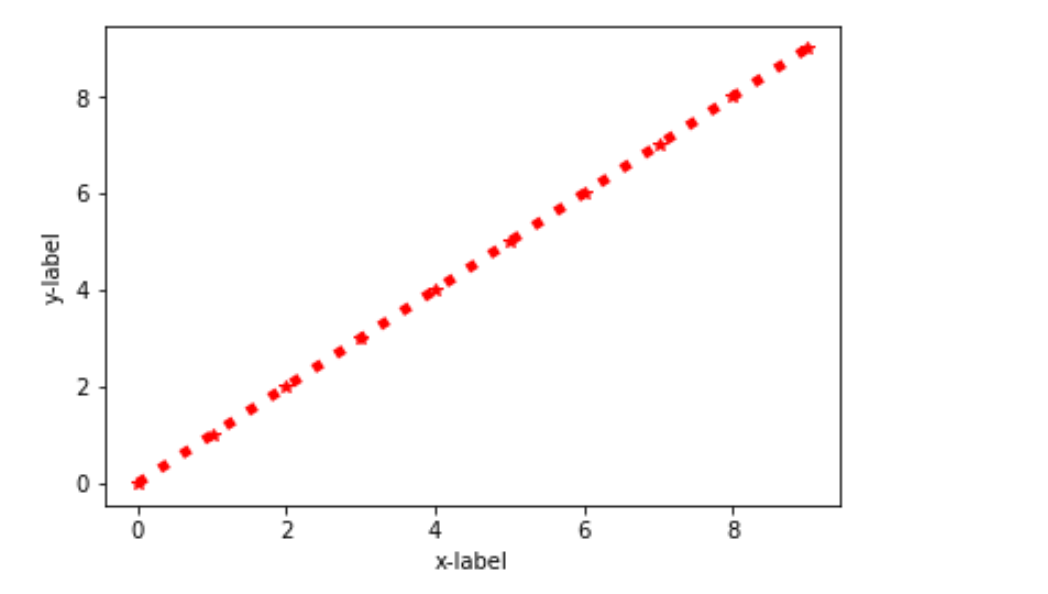说明：Matplotlib默认情况下不支持中文，可以使用rcParams显示中文

 参数 字体样式 'Fangsong' 仿宋体 'STSong' 华文宋体 'SimHei' 黑体 'STKaiti' 华文楷体 'STLiti' 华文隶书

说明：字体样式列举不全

import matplotlib.pyplot as plt
import numpy as np
# 设置字体为华文隶书 显示中文
plt.rcParams['font.sans-serif']=['STLiti']

x=np.arange(0,10)

plt.plot(x,marker='*',linestyle=':',color='r',linewidth=4.5)
# 使用fontsize设置字体大小
plt.xlabel('x轴',fontsize=20)
plt.ylabel('y轴',fontsize=20)

plt.show()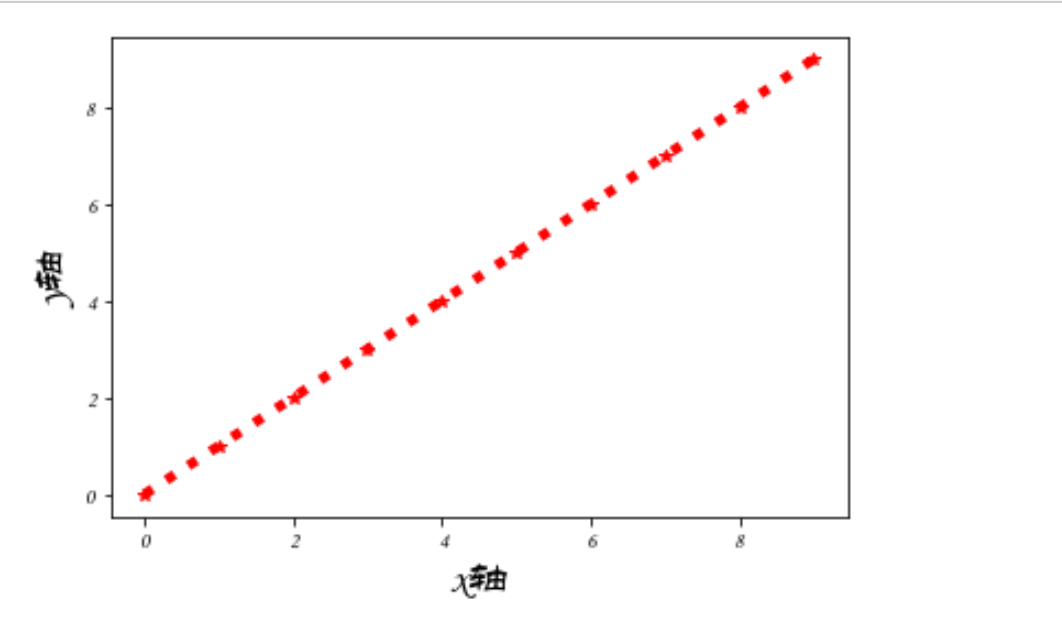### （5）Matplotlib标题使用title()方法

import matplotlib.pyplot as plt
import numpy as np
# 设置字体为华文隶书 显示中文
plt.rcParams['font.sans-serif']=['STLiti']

x=np.arange(0,10)

plt.plot(x,marker='*',linestyle=':',color='r',linewidth=4.5)
# fontsize设置字体大小
plt.xlabel('x轴',fontsize=20)
plt.ylabel('y轴',fontsize=20)
plt.title('标题',fontsize=25)
plt.show()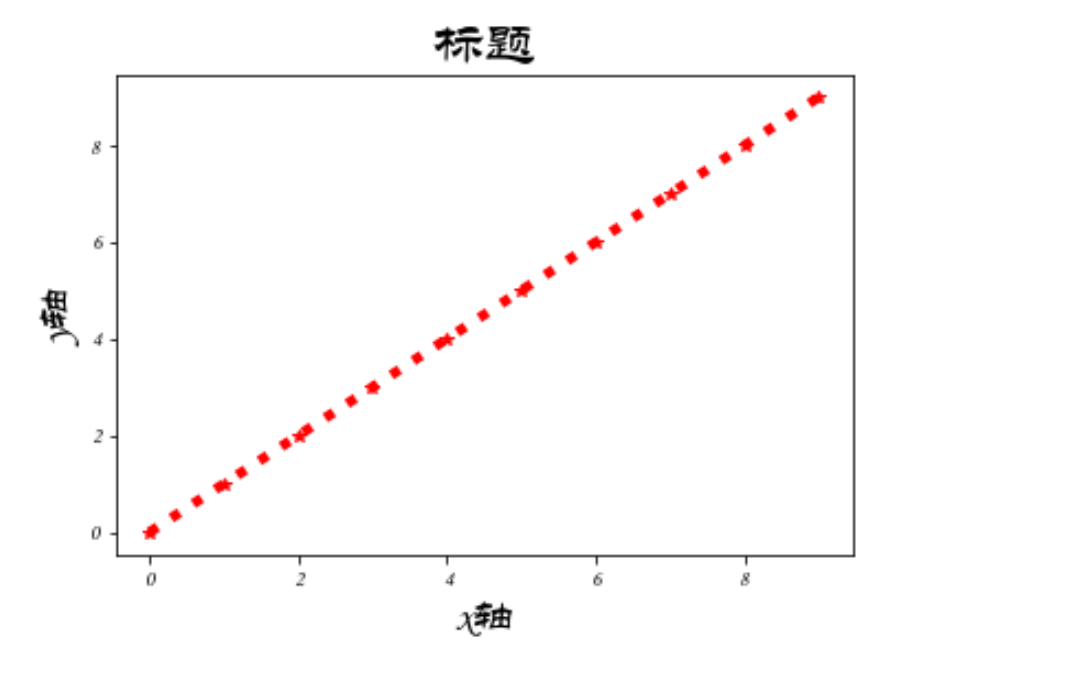### （6）Matplotlib标题与标签的定位提供了loc参数

 方法 参数 默认 title() 'left', 'right', 和 'center' 'center' xlabel() 'left', 'right', 和 'center' 'center' ylabel() 'bottom', 'top', 和 'center' 'center'
import matplotlib.pyplot as plt
import numpy as np

# 设置字体为黑体 显示中文
plt.rcParams['font.sans-serif']=['STLiti']

x=np.arange(0,10)

plt.plot(x,marker='o',color='r',linewidth=2)

# fontsize设置字体大小
# loc设置标签显示位置
plt.xlabel('x轴',fontsize=20,loc='left')
plt.ylabel('y轴',fontsize=20,loc='top')
plt.title('标题',fontsize=25,loc="left")
plt.show()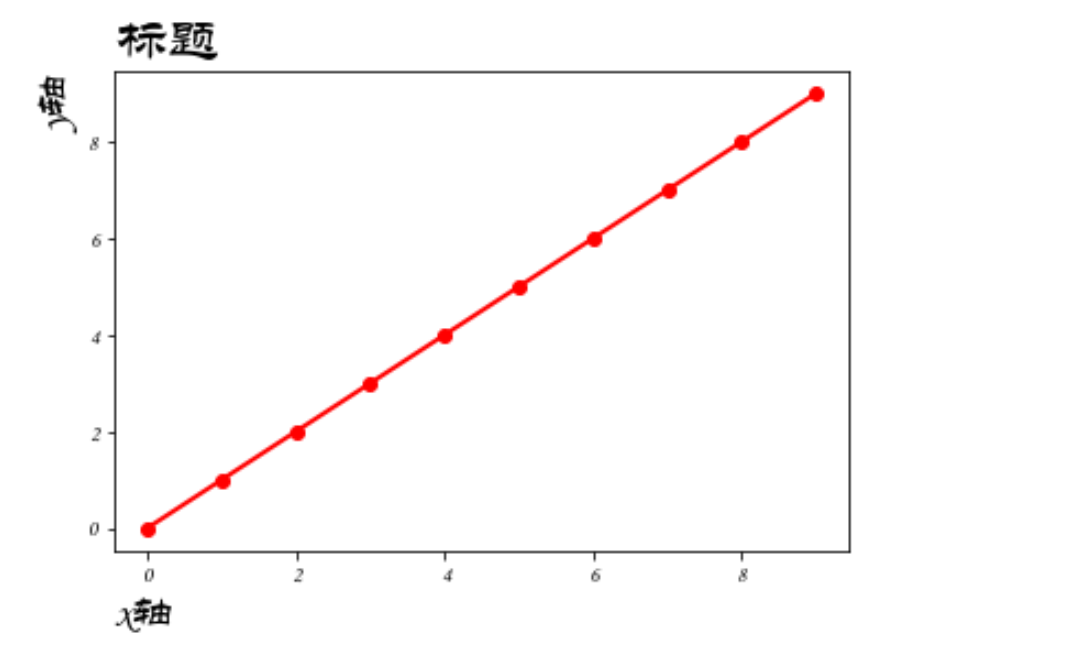说明：最开始我试的时候，标签一直显示没有loc参数。所以用了另一种方法，用到了rcParams（标签有loc参数的请忽略

import matplotlib.pyplot as plt
import numpy as np

# 设置字体为黑体 显示中文
plt.rcParams['font.sans-serif']=['STLiti']

plt.rcParams['xaxis.labellocation']='right'
plt.rcParams['yaxis.labellocation']='top'

x=np.arange(0,10)

plt.plot(x,marker='o',color='r',linewidth=2)

# fontsize设置字体大小
# loc设置标签显示位置
plt.xlabel('x轴',fontsize=20)
plt.ylabel('y轴',fontsize=20)
plt.title('标题',fontsize=25,loc='right')
plt.show()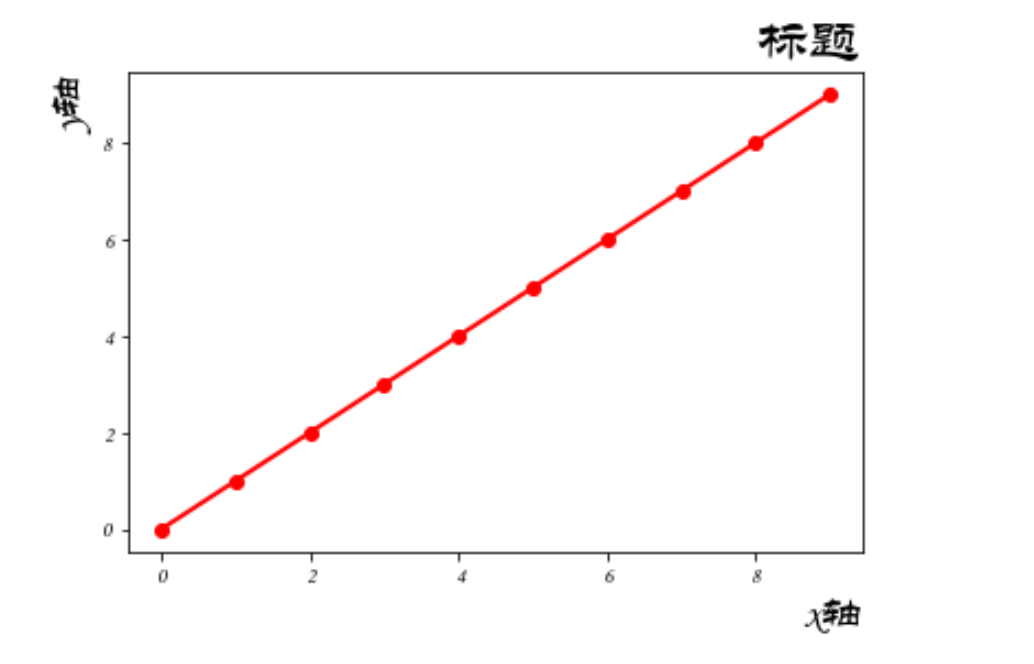### （7）Matplotlib网格线使用pyplot中的grid()方法

grid()语法：

matplotlib.pyplot.grid(b=None, which='major', axis='both', )

• b：可选，默认为 None，可以设置布尔值，true 为显示网格线，false 为不显示，如果设置 **kwargs 参数，则值为 true。
• which：可选，可选值有 'major'、'minor' 和 'both'，默认为 'major'，表示应用更改的网格线。
• axis：可选，设置显示哪个方向的网格线，可以是取 'both'（默认），'x' 或 'y'，分别表示两个方向，x 轴方向或 y 轴方向。
• **kwargs：可选，设置网格样式，可以是 color='r', linestyle='-' 和 linewidth=2，分别表示网格线的颜色，样式和宽度。

例1：添加网格线，参数使用默认值。plt.grid()

import matplotlib.pyplot as plt
import numpy as np

# 设置字体为黑体 显示中文
plt.rcParams['font.sans-serif']=['STLiti']

x=np.arange(0,10)

plt.plot(x,marker='o',color='r',linewidth=2)

# 设置标签和标题
# fontsize设置字体大小
# loc设置标签显示位置
plt.xlabel('x轴',fontsize=20,loc='center')
plt.ylabel('y轴',fontsize=20,loc='center')
plt.title('标题',fontsize=25,loc='center')

# 设置网格线
plt.grid()

plt.show()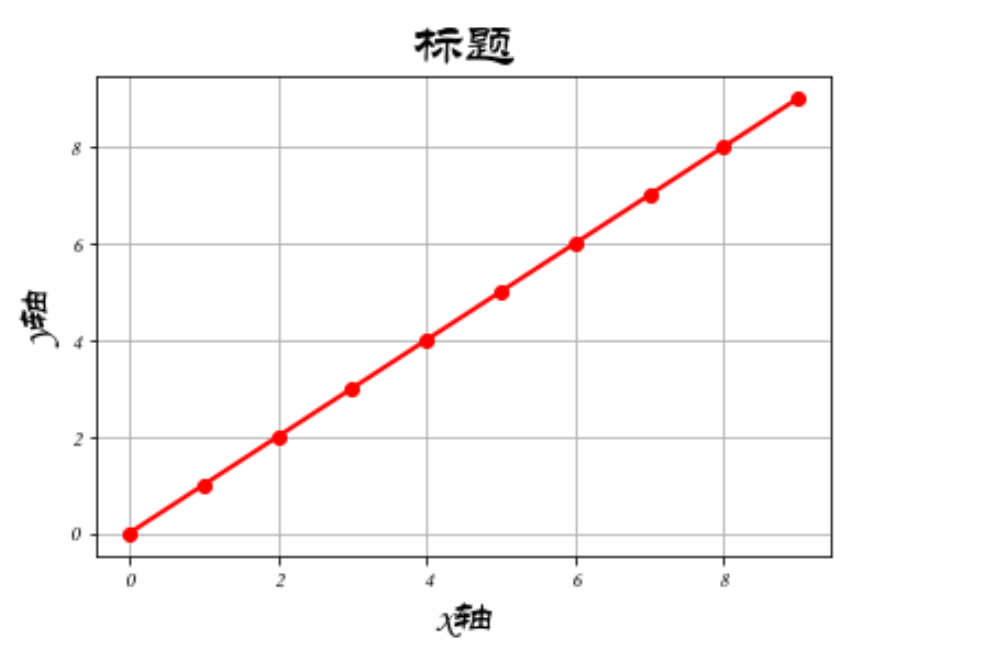例2：使用axis参数控制网格线显示方向。plt.grid(axis='x')或者plt.grid(axis='y')

import matplotlib.pyplot as plt
import numpy as np

# 设置字体为黑体 显示中文
plt.rcParams['font.sans-serif']=['STLiti']

x=np.arange(0,10)

plt.plot(x,marker='o',color='r',linewidth=2)

# 设置标签和标题
# fontsize设置字体大小
# loc设置标签显示位置
plt.xlabel('x轴',fontsize=20,loc='center')
plt.ylabel('y轴',fontsize=20,loc='center')
plt.title('标题',fontsize=25,loc='center')

# 设置网格线
# axis='x' 设置在x轴方向显示网格线 设置y就在y轴方向显示网格线
plt.grid(axis='x')

plt.show()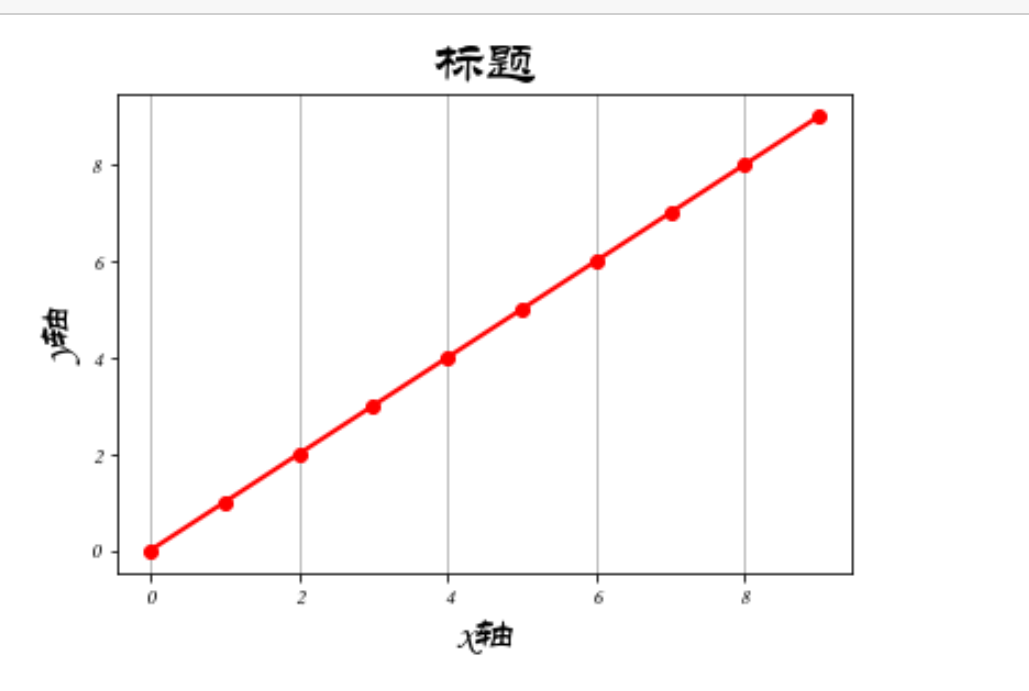例3：更改网格线样式 参数color,linestyle,linewidth等同于Matplotlib绘图线

import matplotlib.pyplot as plt
import numpy as np

# 设置字体为黑体 显示中文
plt.rcParams['font.sans-serif']=['STLiti']

x=np.arange(0,10)

plt.plot(x,marker='o',color='r',linewidth=2)

# 设置标签和标题
# fontsize设置字体大小
# loc设置标签显示位置
plt.xlabel('x轴',fontsize=20,loc='center')
plt.ylabel('y轴',fontsize=20,loc='center')
plt.title('标题',fontsize=25,loc='center')

# 设置网格线
# axis='x' 设置在x轴方向显示网格线 设置y就在y轴方向显示网格线
# color='g'设置网格线颜色
# linestyle='-.'设置网格线样式
# linewidth=2设置网格线宽度
plt.grid(axis='x',color='g',linestyle='-.',linewidth=2)

plt.show()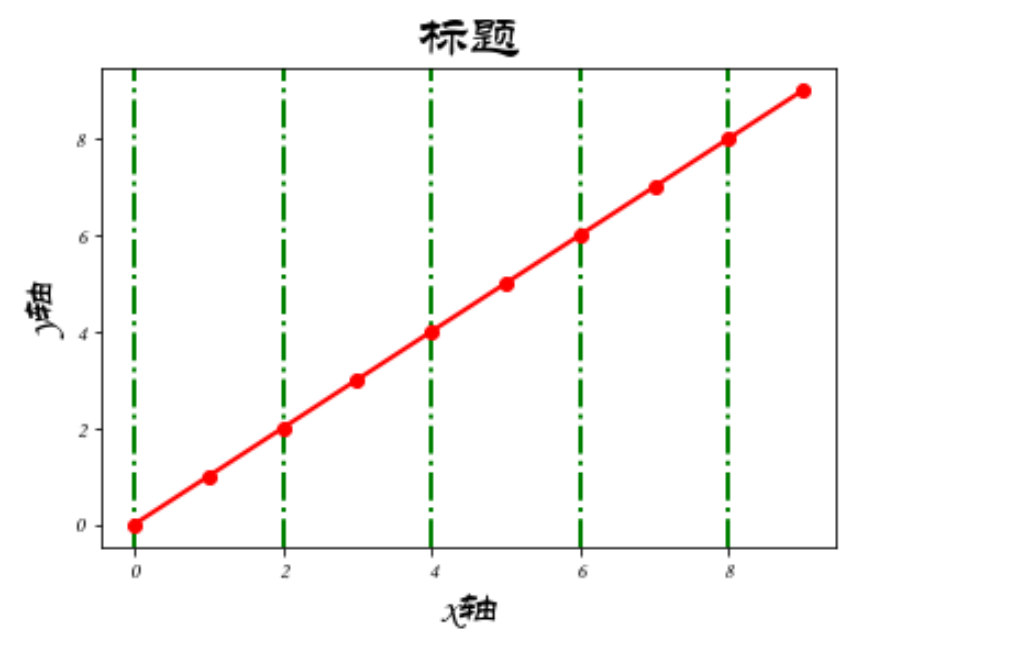### （8）绘制多图使用pyplot中的subplot()和subplots()方法

两者区别：

subplot() 方法：在绘图时需要指定位置

subplots() 方法：可以一次生成多个，在调用时只需要调用生成对象的 ax 即可。

subplot()实例：

subplot语法：

subplot(nrows, ncols, index, **kwargs)
• nrows：默认为 1，设置图表的行数。
• ncols：默认为 1，设置图表的列数。
• index：默认为1，设置图表的编号。
import matplotlib.pyplot as plt
import numpy as np

# plot1
xplot1=np.array([0,6])
yplot1=np.array([0,100])
# plt.subplot()中的三个参数分成nrows(行)、ncols(列)和index(编号)
plt.subplot(2,2,1)  # 两行两列 第一个图
plt.plot(xplot1,yplot1,color='g',linestyle='--',linewidth=2)
# 小标题
plt.title('plot 1',fontsize=20)

# plot2
xplot2=np.array([1,2,3,4,5])
yplot2=np.array([1,4,9,16,25])
plt.subplot(2,2,2)  # 两行两列 第二个图
plt.plot(xplot2,yplot2,color='r',linestyle=':',linewidth=2)
# 小标题
plt.title('plot 2',fontsize=20)

# plot3
xplot3=np.array([1,2,3,4,5])
yplot3=np.array([1,4,9,16,25])
plt.subplot(2,2,3)  # 两行两列 第三个图
plt.plot(xplot3,yplot3,color='b',linestyle='-.',linewidth=2)
# 小标题
plt.title('plot 3',fontsize=20)

# plot4
xplot4=np.array([1,2,3,4,5])
yplot4=np.array([1,4,9,16,25])
plt.subplot(2,2,4)  # 两行两列 第四个图
plt.plot(xplot4,yplot4,color='y',linestyle='-',linewidth=2)
# 小标题
plt.title('plot 4',fontsize=20)

# 总标题
plt.suptitle('plots',fontsize=20)

plt.show()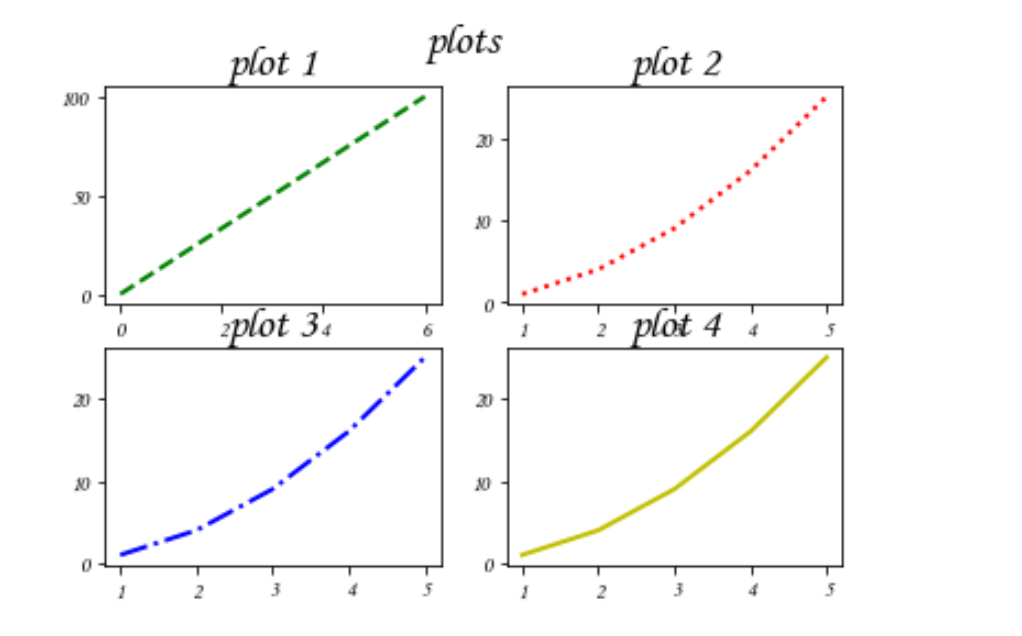subplots()实例：

subplots()语法：

matplotlib.pyplot.subplots(nrows=1, ncols=1, *, sharex=False, sharey=False, squeeze=True, subplot_kw=None, gridspec_kw=None, **fig_kw)
• nrows：默认为 1，设置图表的行数。
• ncols：默认为 1，设置图表的列数。
• sharex、sharey：设置 x、y 轴是否共享属性，默认为 false，可设置为 'none'、'all'、'row' 或 'col'。 False 或 none 每个子图的 x 轴或 y 轴都是独立的，True 或 'all'：所有子图共享 x 轴或 y 轴，'row' 设置每个子图行共享一个 x 轴或 y 轴，'col'：设置每个子图列共享一个 x 轴或 y 轴。
• squeeze：布尔值，默认为 True，表示额外的维度从返回的 Axes(轴)对象中挤出，对于 N*1 或 1*N 个子图，返回一个 1 维数组，对于 N*M，N>1 和 M>1 返回一个 2 维数组。如果设置为 False，则不进行挤压操作，返回一个元素为 Axes 实例的2维数组，即使它最终是1x1。
• gridspec_kw：可选，字典类型。把字典的关键字传递给 GridSpec 构造函数创建子图放在网格里(grid)。
• **fig_kw：把详细的关键字参数传给 figure() 函数。
import matplotlib.pyplot as plt
import numpy as np

# numpy.linspace作用为：在指定的大间隔内，返回固定间隔的数据(可以理解为等差数列)
# numpy.linspace语法:numpy.linspace(start, stop, num=50, endpoint=True, retstep=False, dtype=None,axis=None)
# start:起始点
# stop:结束点
# num:生成的样本数（默认50，必须是非负）
# endpoint:默认为True（为False时，不输出结束点）
# retstep默认为False（为True时,返回每个步骤之间的间距）(可以理解为等差数列的公差)
# dtype:输出数组的类型
# axis=0时，输出最开始的轴值，axis=-1时，输出最后的轴值

# np.pi是一个常数表示圆周率Π
# 2*np.pi是2 x Π
x=np.linspace(0,2*np.pi,400)
y=np.sin(x**2)

# 创建一个画像和子图 -----图1
# fig代表绘图窗口(Figure)
# fig=plt.figure()
# figure()语法:figure(num=None, figsize=None, dpi=None, facecolor=None, edgecolor=None, frameon=True)
# num:图像编号或名称，数字为编号 ，字符串为名称
#figsize:指定figure的宽和高，单位为英寸；
#dpi参数指定绘图对象的分辨率，即每英寸多少个像素，缺省值为80 ,1英寸等于2.5cm,A4纸是 21*30cm的纸张
#facecolor:背景颜色
#edgecolor:边框颜色
#frameon:是否显示边
# ax代表这个绘图窗口上的坐标系(axis)
fig,ax=plt.subplots()
ax.plot(x,y)
ax.set_title('first')

# 创建两个子图   -----图2
# subplots语法:(nrows=1, ncols=1, *, sharex=False, sharey=False, squeeze=True, subplot_kw=None, gridspec_kw=None,
# **fig_kw)
# nrows：默认为 1，设置图表的行数。
# ncols：默认为 1，设置图表的列数。
# sharex、sharey：设置 x、y 轴是否共享属性，默认为 false，可设置为 'none'、'all'、'row' 或 'col'。 False 或 none 每个子图的 x 轴或 y 轴都是独立的，True 或 'all'：所有子图共享 x 轴或 y 轴，'row' 设置每个子图行共享一个 x 轴或 y 轴，'col'：设置每个子图列共享一个 x 轴或 y 轴。
# squeeze：布尔值，默认为 True，表示额外的维度从返回的 Axes(轴)对象中挤出，对于 N*1 或 1*N 个子图，返回一个 1 维数组，对于 N*M，N>1 和 M>1 返回一个 2 维数组。如果设置为 False，则不进行挤压操作，返回一个元素为 Axes 实例的2维数组，即使它最终是1x1。
# gridspec_kw：可选，字典类型。把字典的关键字传递给 GridSpec 构造函数创建子图放在网格里(grid)。
# **fig_kw：把详细的关键字参数传给 figure() 函数。

# 创建一个画像和两个子图  一行两列 共享y轴
f,(ax1,ax2)=plt.subplots(1,2,sharey=True)
ax1.plot(x,y)
ax1.set_title('one')
# scatter(x,y) 在向量 x 和 y 指定的位置创建一个散点图。该类型的图形也称为气泡图。
ax2.scatter(x,y)
ax2.set_title('two')

# 创建四个子图   -----图3
# projection='polar'等价于polar=True 极坐标图投影
fig,axs=plt.subplots(2,2,subplot_kw=dict(projection='polar'))
# [0,0],[1,1]散射点
axs[0,0].plot(x,y)
axs[1,1].scatter(x,y)

# 共享x轴
# axs.subplots(2,2,sharex='col')

# 共享y轴
# plt.subplots(2,2,sharey='row')

# 共享x轴和y轴 第一种
# plt.subplots(2,2,sharex='col',sharey='row')

# 共享x轴和y轴 第二种   ------图4
plt.subplots(2,2,sharex=True,sharey=True)

plt.show()

效果如图：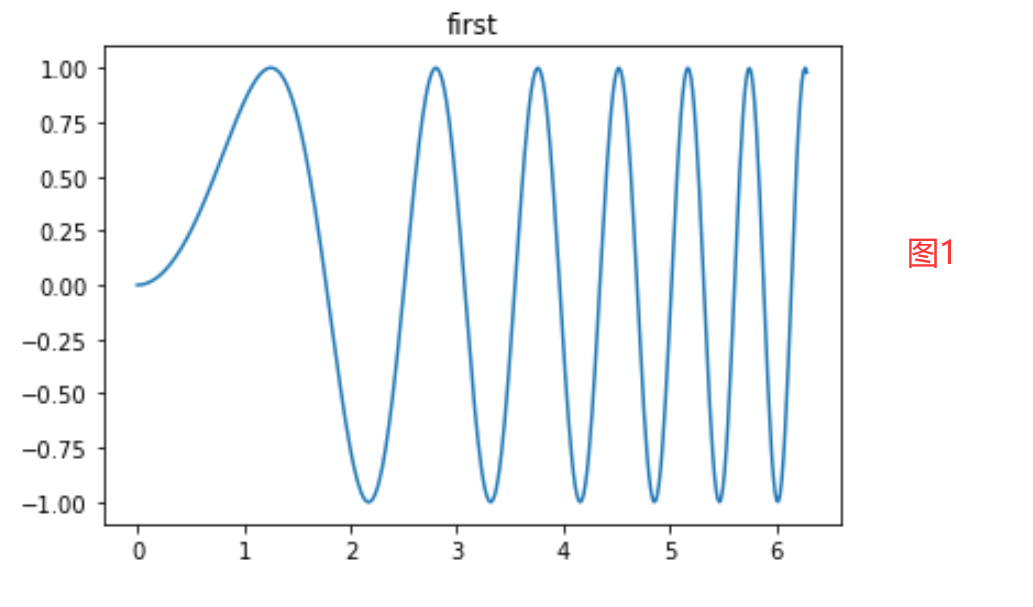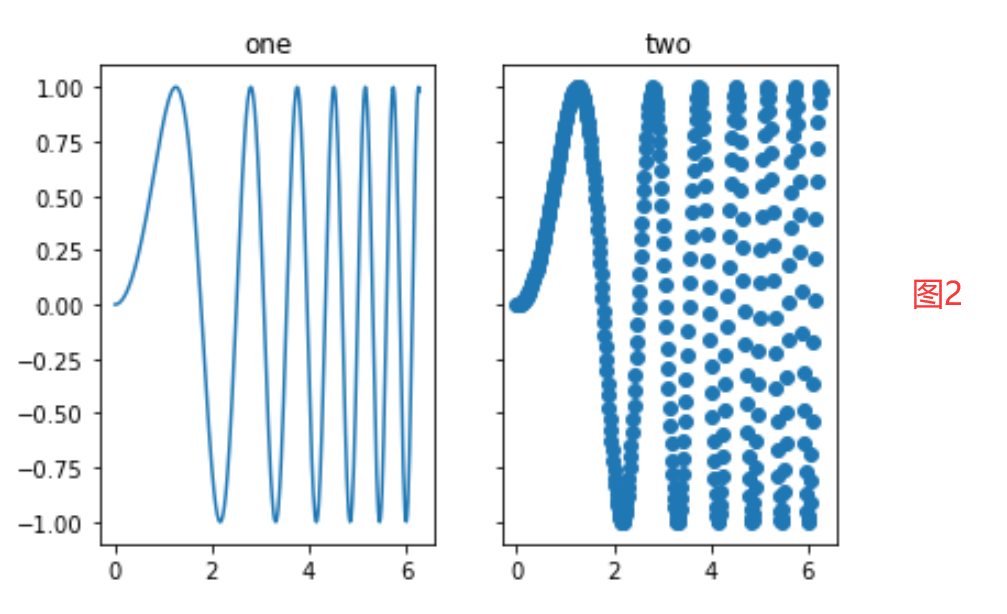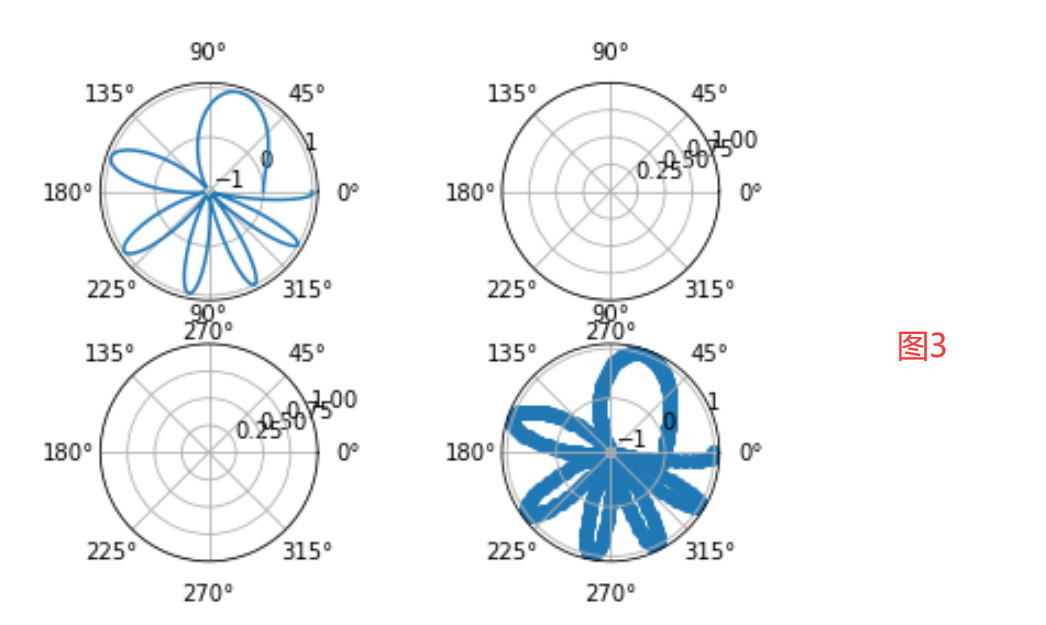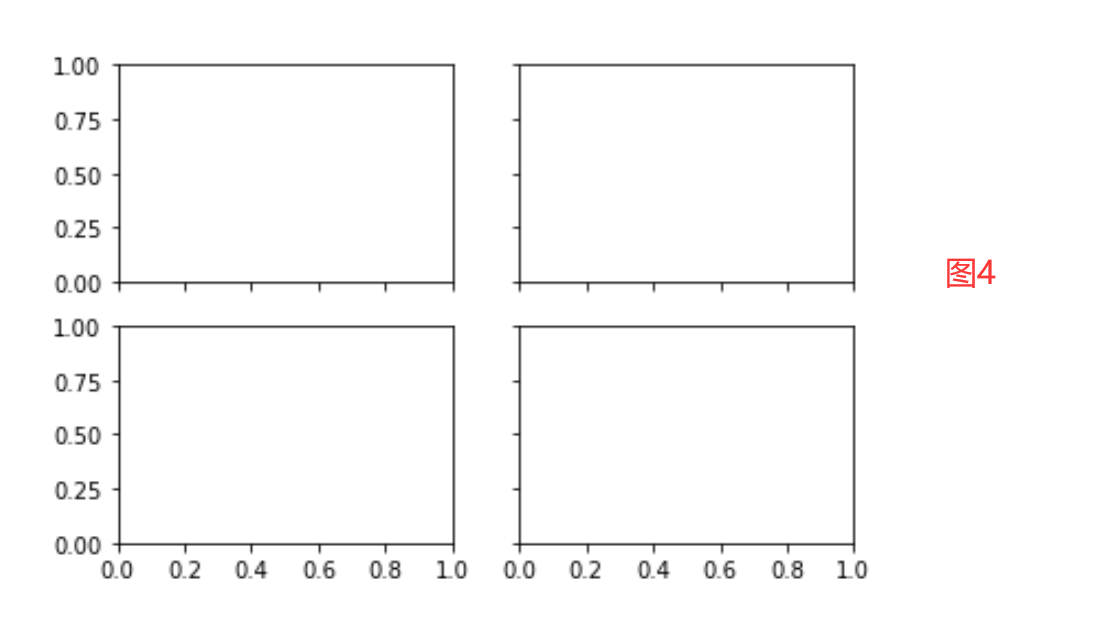展开全文python
• @PyQt5记录笔记之使用Matplotlib绘图 PyQt5上创建Matplotlib绘图的基本流程 1，要在PyQt5界面上显示，首先要创建界面组件FigureCanvas（相当于用于显示Matplotlib的Widget组件）； 2，接着需要创建绘图相关组件...
• 案例包括点击画图功能，和显示 matplotlib 导航条功能（比如，显示保存、放大等功能按钮）；但是，matplotlib 自带的导航条功能是英文的，做软件时用户起来可能不方便。
• 使用matplotlib绘图，标题或坐标等设置中文时，会遇到显示成方框形的问题，对此，需要通过修改matplotlib字体或者直接在代码中设置指定中文字体来解决这个问题 解决方法有两类：永久生效、临时设置 参考资料 ...python jupyter
• Matplotlib进行绘图操作 配置 Python 2.7 或 3.6 Numpy 1.13.3 Matplotlib 2.0.0 简介 Matplotlab是python最著名的绘图库，它提供了一整套和matlab相似的命令API,十分适合交互式地制图，其中，matplotlib地pyplot...
• 来自<Python成为专业人士笔记系列>python成为专业人士笔记​biblog....它利用通用的图形用户界面工具包，如Tkinter, wxPython, Qt或GTK+，向应用程序嵌入式绘图提供了应用程序接口（API）。此外，matplotl...
• 利用ipython --pylab，可以进入PyLab模式，已经导入了matplotlib库与相关软件包（例如Numpy和Scipy)，额可以直接使用相关库的功能。 本文作为学习过程中对matplotlib一些常用知识点的整理，方便查找。 这样IPython...
• 1、查看matplotlib配置文件的位置 import matplotlib print(matplotlib.matplotlib_fname()) /home/kiosk/anaconda3/lib/python3.7/site-packages/matplotlib/mpl-data/matplotlibrc 2、将字体放在指定目录 SimHei....
• 今天小编就为大家分享一篇完美解决Python matplotlib绘图时汉字显示不正常的问题，具有很好的参考价值，希望对大家有所帮助。一起跟随小编过来看看吧
• ## pyqt5+matplotlib绘图

千次阅读 2021-03-12 16:29:45
1. 整体界面设计 ...4. graphicsView：绘图的一个界面（后面将使用matplotlib的图层进行替换，这里是为了方便预览和设计） 代码： from PyQt5 import QtCore, QtGui, QtWidgets class Ui_MainWinpython pyqt5
• 使用python的matplotlib绘图时出现图例无法显示中文的情况。 2.解决方案 在plt.figure()中添加下面两行代码即可解决，原因应该是字体安装的问题。 plt.rcParams['font.sans-serif']=['SimHei'] #显示中文标签 ...
• 使用matplotlib.use('TkAgg')解决导出图为空图层的问题（jupyter notebook）
• 主要介绍了在pycharm中使用matplotlib.pyplot 绘图时报错的解决，具有很好的参考价值，希望对大家有所帮助。一起跟随小编过来看看吧
•   使用matplotlib绘图的原理，主要就是理解figure(画布)、axes(坐标系)、axis(坐标轴)三者之间的关系。   以“美院学生张三写生画画”为例，来说明这三者之间的关系。   首先，张三想要画画，是不是需要在画板...matplotlib
• 提示：文章写完后，目录可以...`毕业设计要用到深度学习，于是想着使用docker+pycharm来搭建深度学习环境，使用过程中遇到一个问题，代码中使用matplotlib，无法将图片直接绘制出来，报错，有一个tensorboardx的解.python docker pytorch
• pandas读取csv,excel等数据，然后使用matplotlib绘出其中图像（包括点状图，直方图，雷达图等等）
• ## Matplotlib绘图笔记

千次阅读 2022-03-24 12:05:23python...